# 推荐系统！基于tensorflow搭建混合神经网络精准推荐！ ⛵

+关注继续查看💡 作者：韩信子@ShowMeAI
📘 深度学习实战系列https://www.showmeai.tech/tutorials/42
📘 TensorFlow 实战系列https://www.showmeai.tech/tutorials/43
📘 本文地址https://www.showmeai.tech/article-detail/310
📢 声明：版权所有，转载请联系平台与作者并注明出处
📢 收藏ShowMeAI查看更多精彩内容🏆 实战数据集下载（百度网盘）：公众号『ShowMeAI研究中心』回复『实战』，或者点击 这里 获取本文 [基于TensorFlow搭建混合神经网络推荐系统](https://www.showmeai.tech/article-detail/310)MovieLens 电影推荐数据集

ShowMeAI官方GitHubhttps://github.com/ShowMeAI-Hub

• 小数据集为 600 名观众对 9000部电影的 10w 个打分，也包括电影标签特征。
• 大数据集为 280000 名观众对 110w 部电影的 2700w 评分。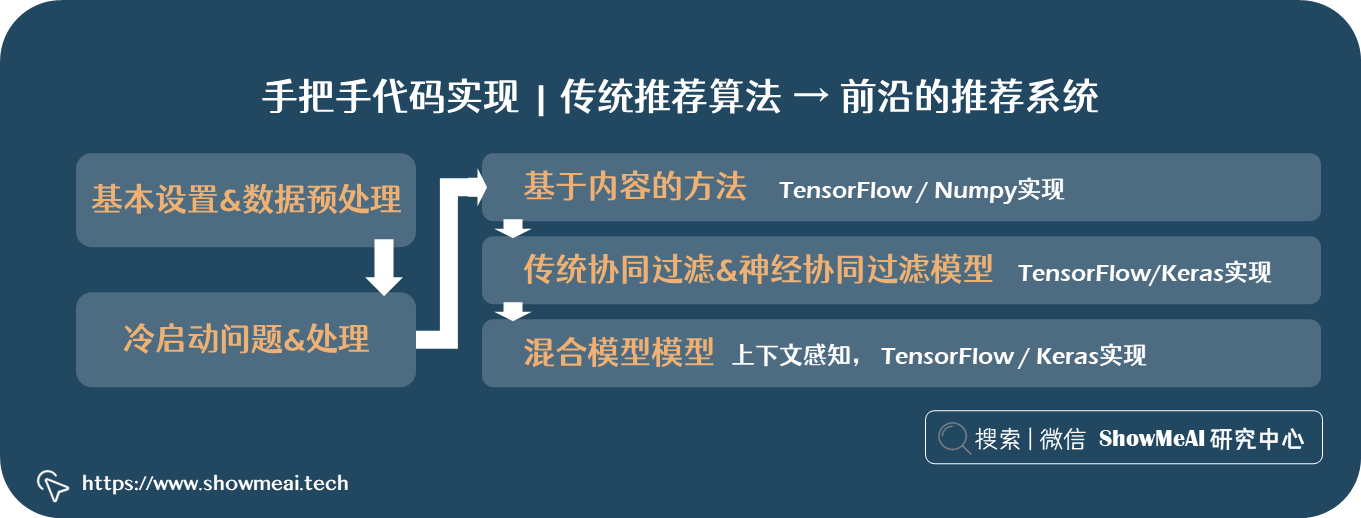• 基本设置&数据预处理
• 冷启动问题&处理
• 基于内容的方法（tensorflow 和 numpy实现）
• 传统协同过滤和神经协同过滤模型（tensorflow/keras 实现）
• 混合模型模型（上下文感知，tensorflow/keras 实现）

# 💡 基本设置&数据预处理

## 📌 工具库导入

# 数据读取与处理
import pandas as pd
import numpy as np
import re
from datetime import datetime

# 绘图
import matplotlib.pyplot as plt
import seaborn as sns

# 评估与预处理
from sklearn import metrics, preprocessing

# 深度学习
from tensorflow.keras import models, layers, utils  #(2.6.0)

## 📌 读取数据

dtf_products = pd.read_csv("movie.csv")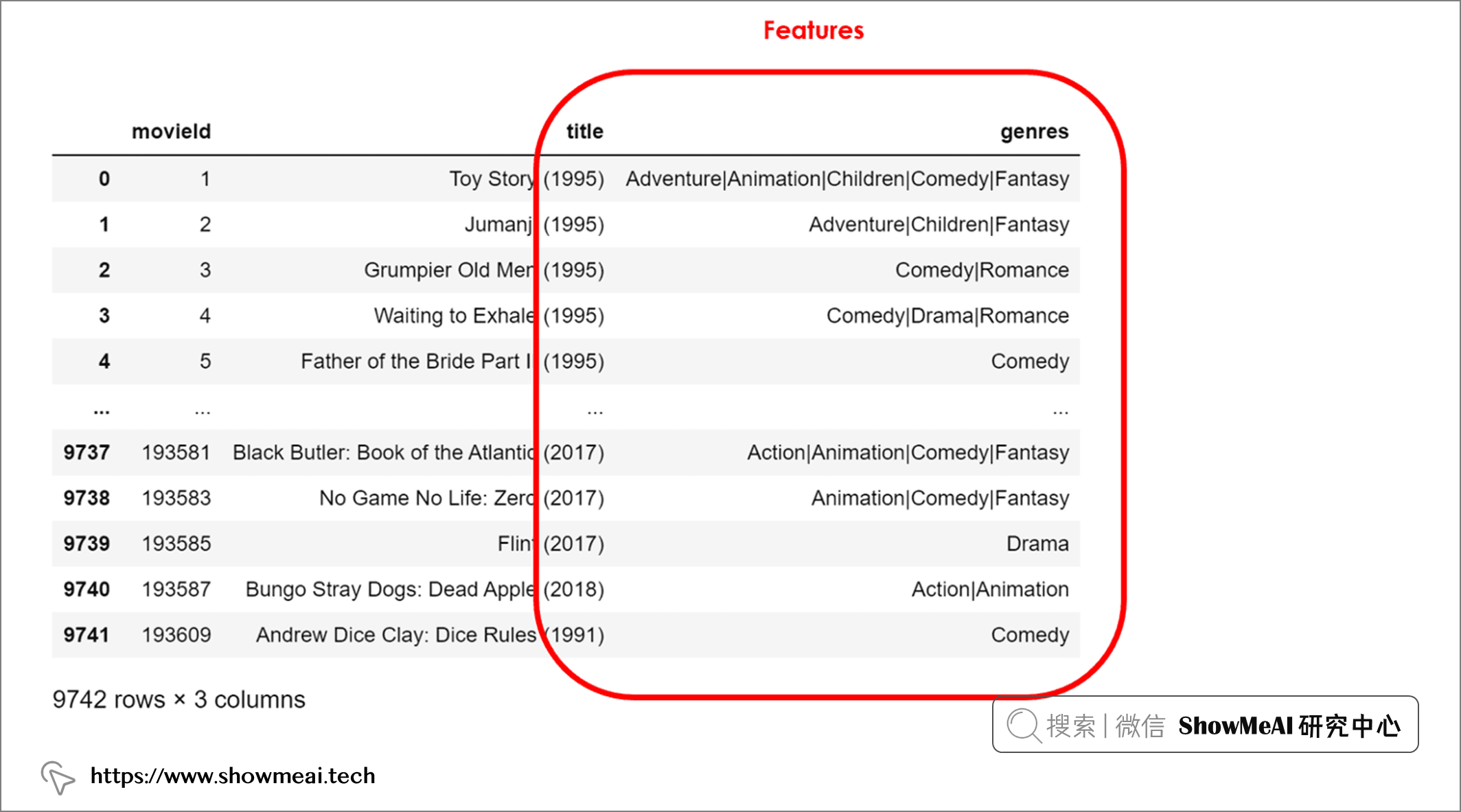movie电影文件中，每一行代表一部电影，右侧的两列包含其特征（标题与题材）。让我们读取用户数据：

dtf_users = pd.read_csv("ratings.csv").head(10000)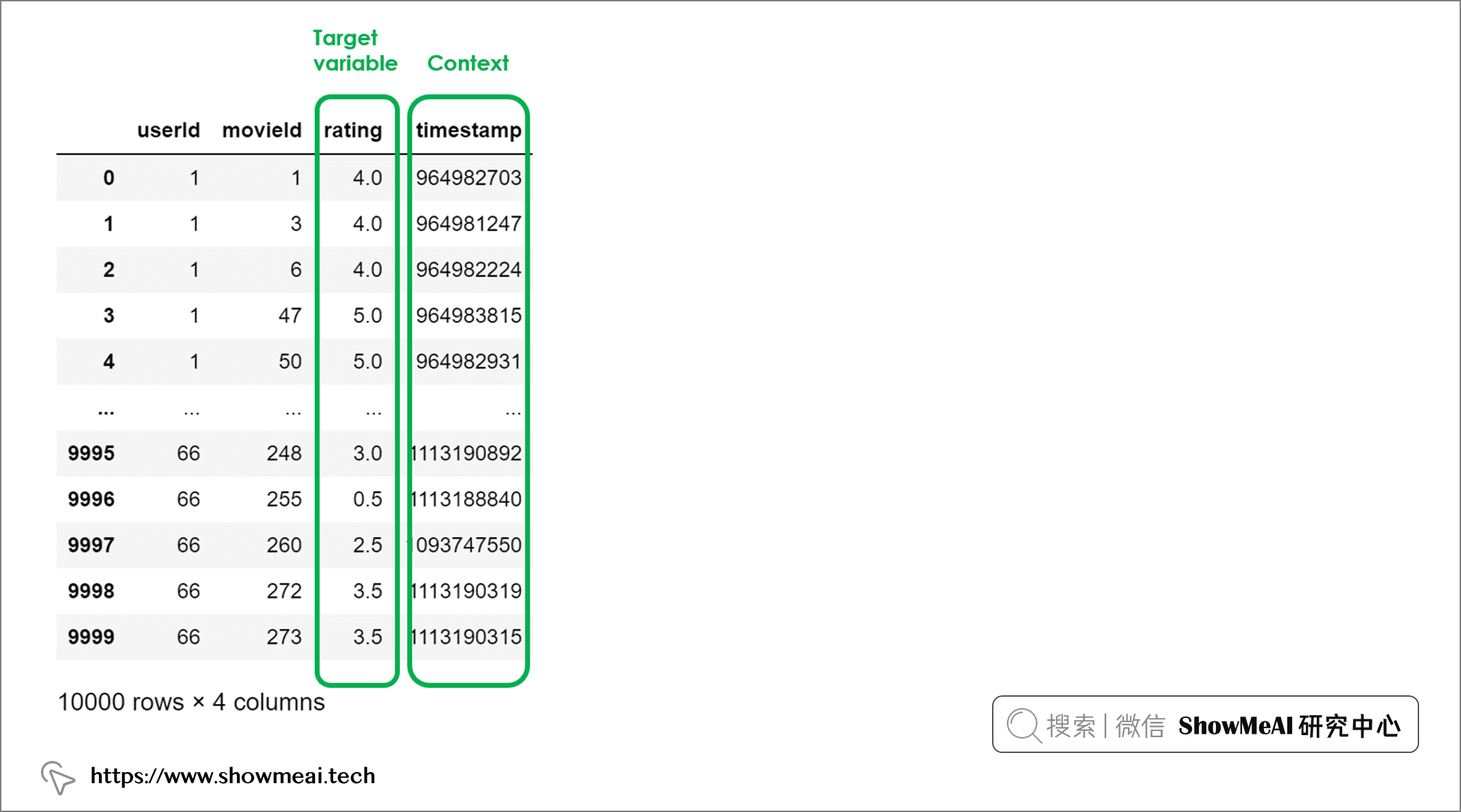## 📌 数据分析&特征工程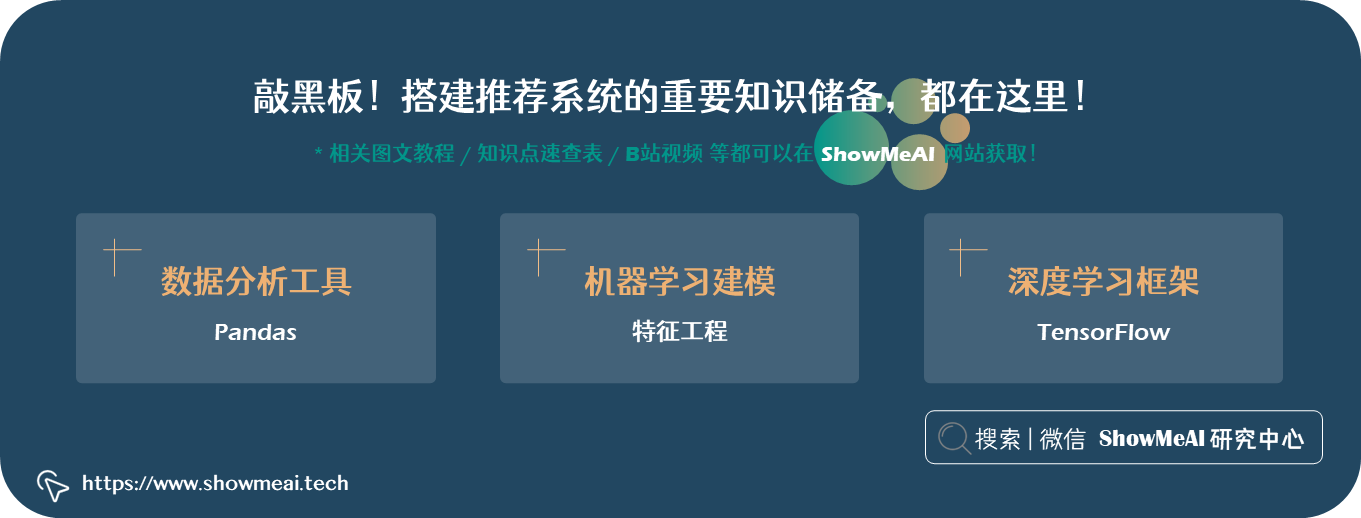📘数据科学工具库速查表 | Pandas 速查表
📘图解数据分析：从入门到精通系列教程
# 电影数据处理
# 题材字段缺失处理
dtf_products = dtf_products[~dtf_products["genres"].isna()]
dtf_products["product"] = range(0,len(dtf_products))
# 电影名称处理
dtf_products["name"] = dtf_products["title"].apply(lambda x: re.sub("[([].*?[)]]", "", x).strip())
# 日期
dtf_products["date"] = dtf_products["title"].apply(lambda x: int(x.split("(")[-1].replace(")","").strip())
if "(" in x else np.nan)
dtf_products["date"] = dtf_products["date"].fillna(9999)
# 判断老电影
dtf_products["old"] = dtf_products["date"].apply(lambda x: 1 if x < 2000 else 0)

# 观众/用户数据处理
dtf_users["user"] = dtf_users["userId"].apply(lambda x: x-1)
dtf_users["timestamp"] = dtf_users["timestamp"].apply(lambda x: datetime.fromtimestamp(x))
# 白天时段
dtf_users["daytime"] = dtf_users["timestamp"].apply(lambda x: 1 if 6<int(x.strftime("%H"))<20 else 0)
# 周末
dtf_users["weekend"] = dtf_users["timestamp"].apply(lambda x: 1 if x.weekday() in [5,6] else 0)

# 电影与用户表合并
dtf_users = dtf_users.merge(dtf_products[["movieId","product"]], how="left")
dtf_users = dtf_users.rename(columns={"rating":"y"})

# 清洗数据
dtf_products = dtf_products[["product","name","old","genres"]].set_index("product")
dtf_users = dtf_users[["user","product","daytime","weekend","y"]]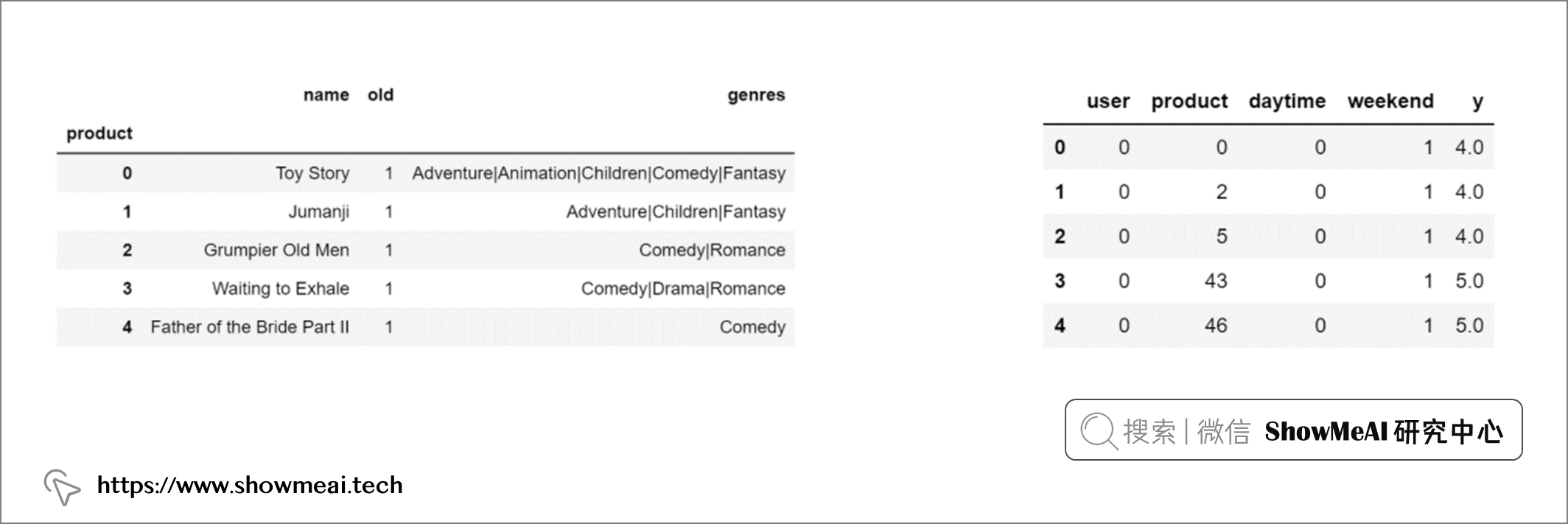dtf_context = dtf_users[["user","product","daytime","weekend"]]

# 电影题材候选统计
tags = [i.split("|") for i in dtf_products["genres"].unique()]
columns = list(set([i for lst in tags for i in lst]))
columns.remove('(no genres listed)')
# 题材可能有多个，切分出来作为标签
for col in columns:
dtf_products[col] = dtf_products["genres"].apply(lambda x: 1 if col in x else 0)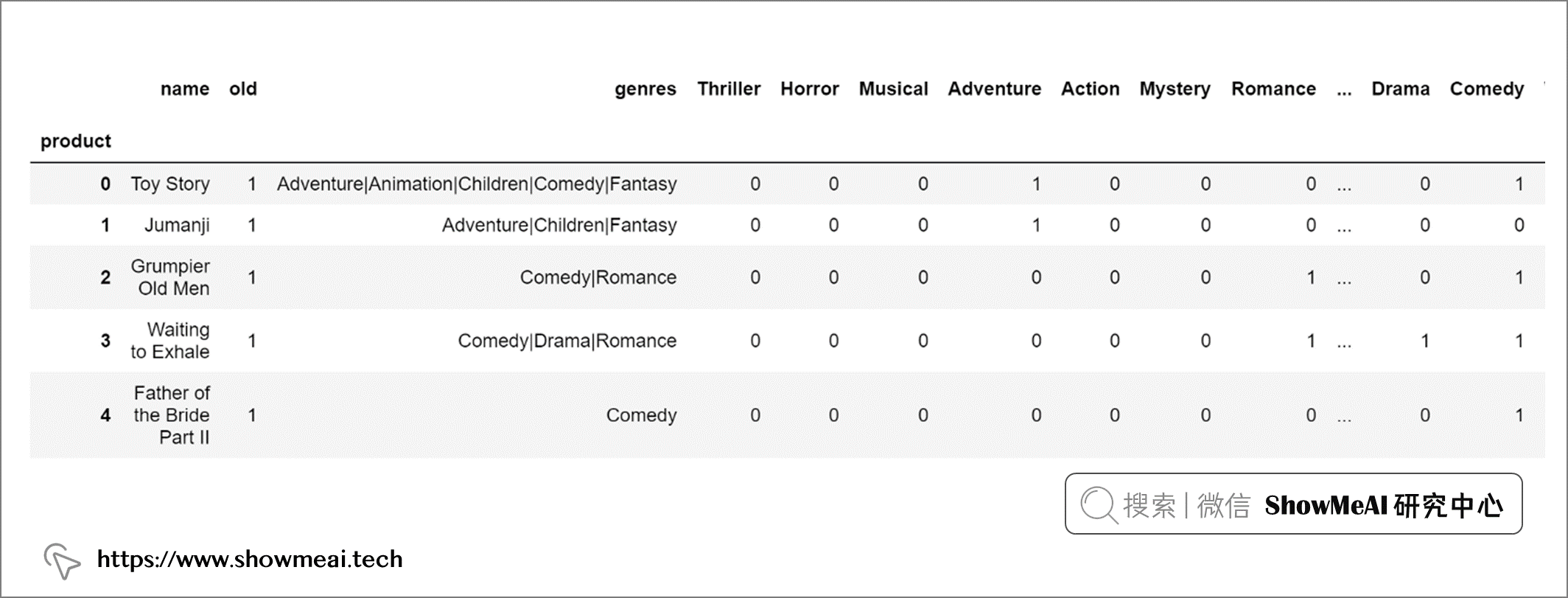# 构建热力图并可视化
fig, ax = plt.subplots(figsize=(20,5))
sns.heatmap(dtf_products==0, vmin=0, vmax=1, cbar=False, ax=ax).set_title("Products x Features")
plt.show()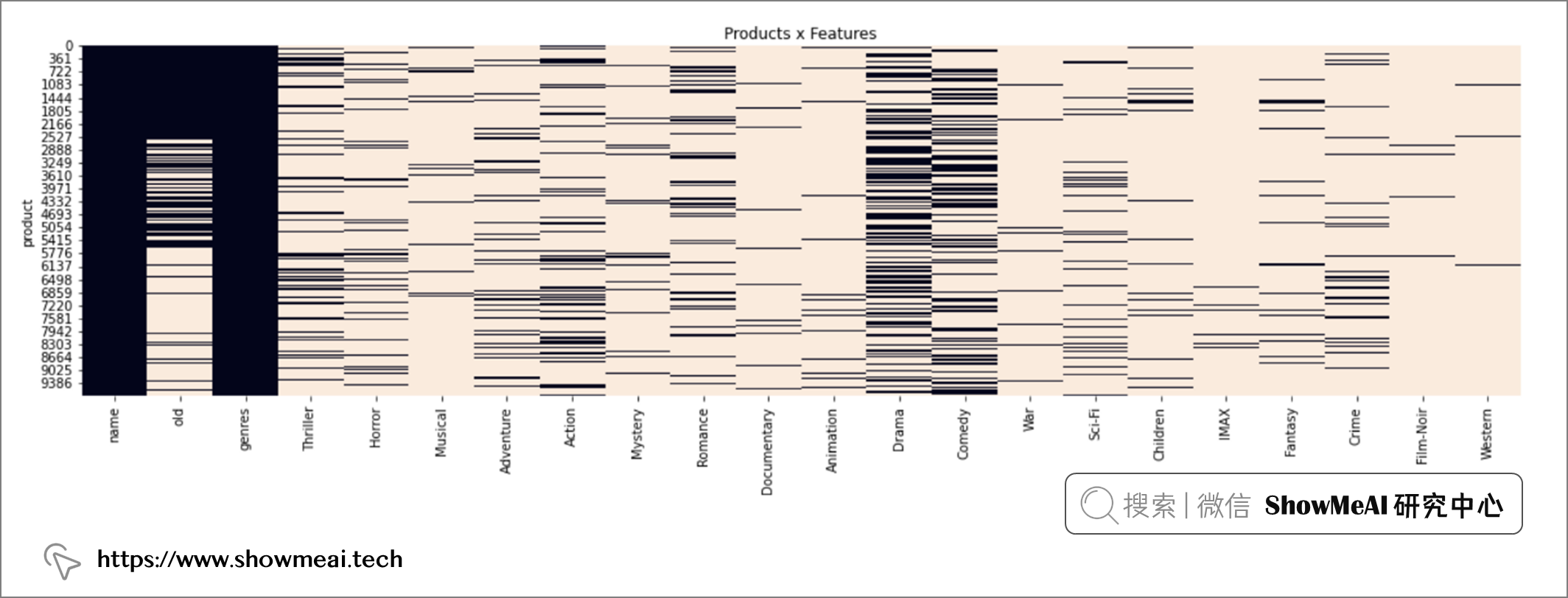tmp = dtf_users.copy()
dtf_users = tmp.pivot_table(index="user", columns="product", values="y")
missing_cols = list(set(dtf_products.index) - set(dtf_users.columns))
for col in missing_cols:
dtf_users[col] = np.nan
dtf_users = dtf_users[sorted(dtf_users.columns)]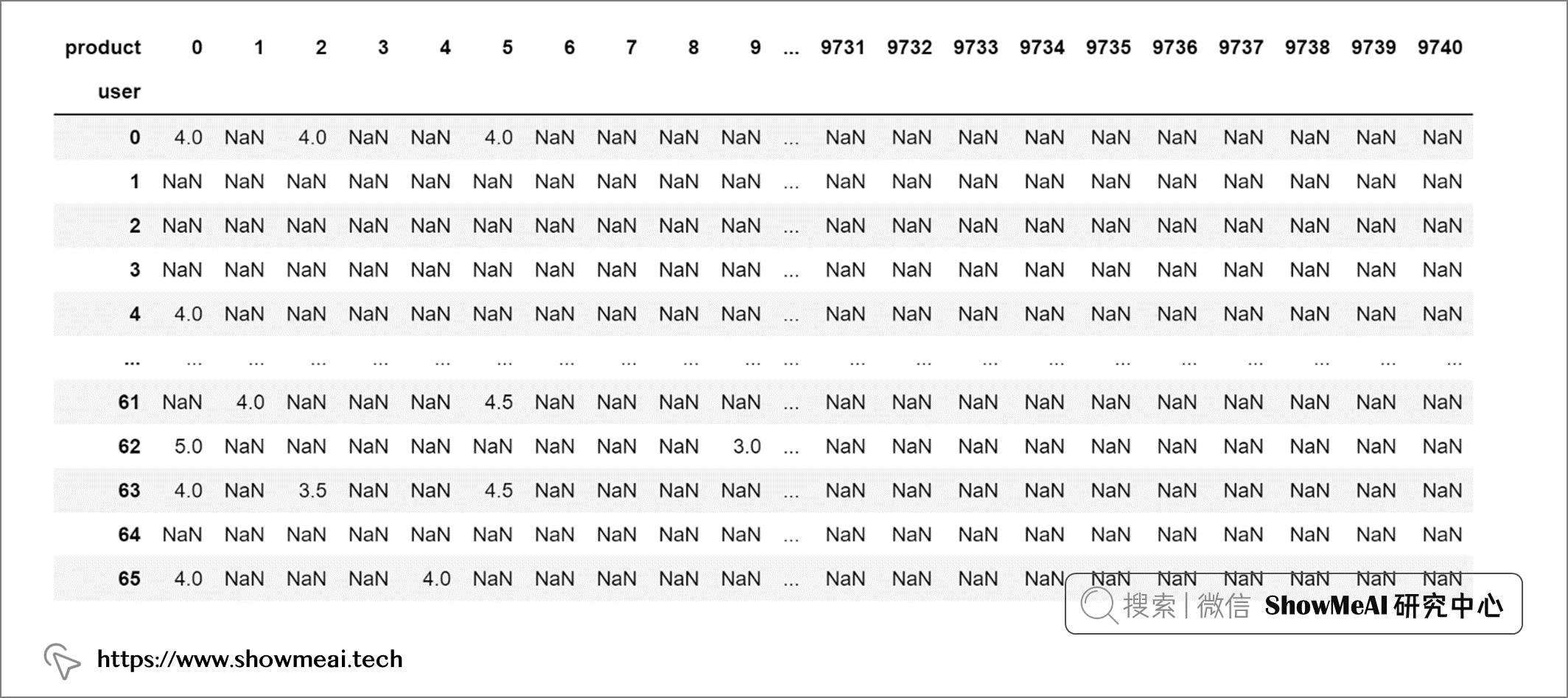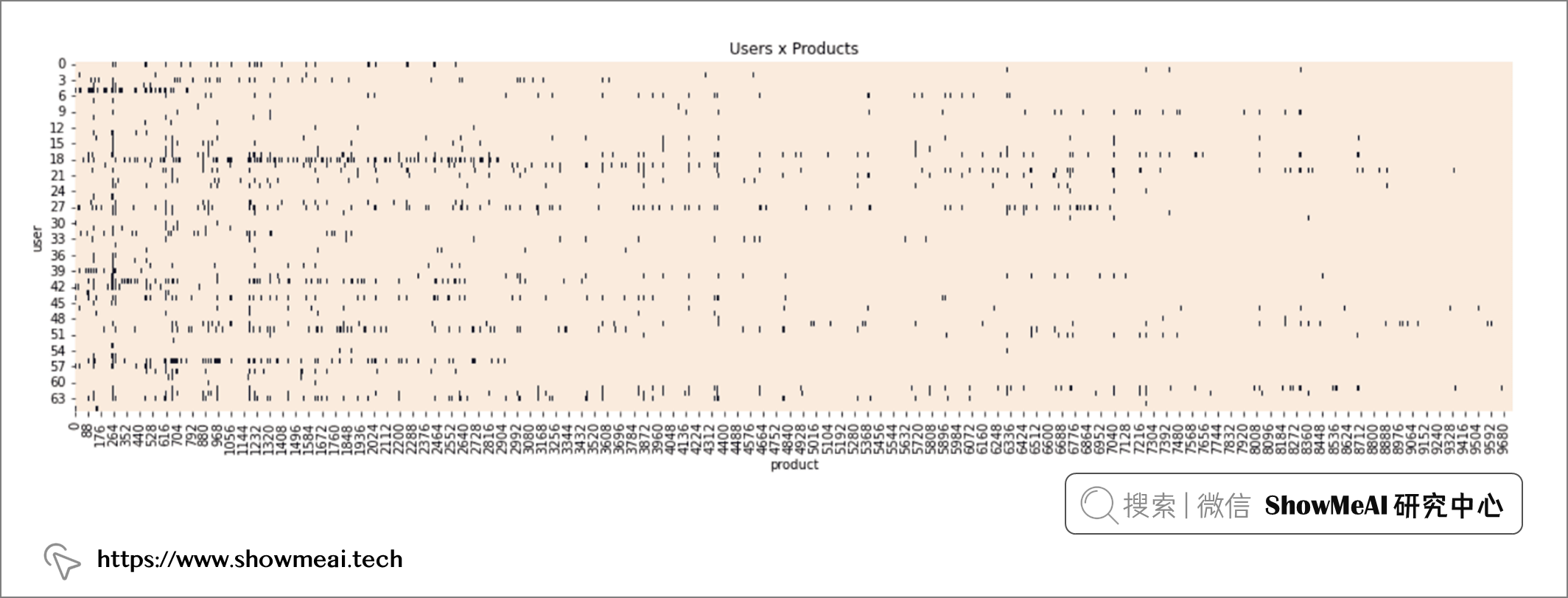# 数据幅度缩放
dtf_users = pd.DataFrame(preprocessing.MinMaxScaler(feature_range=(0.5,1)).fit_transform(dtf_users.values),
columns=dtf_users.columns, index=dtf_users.index)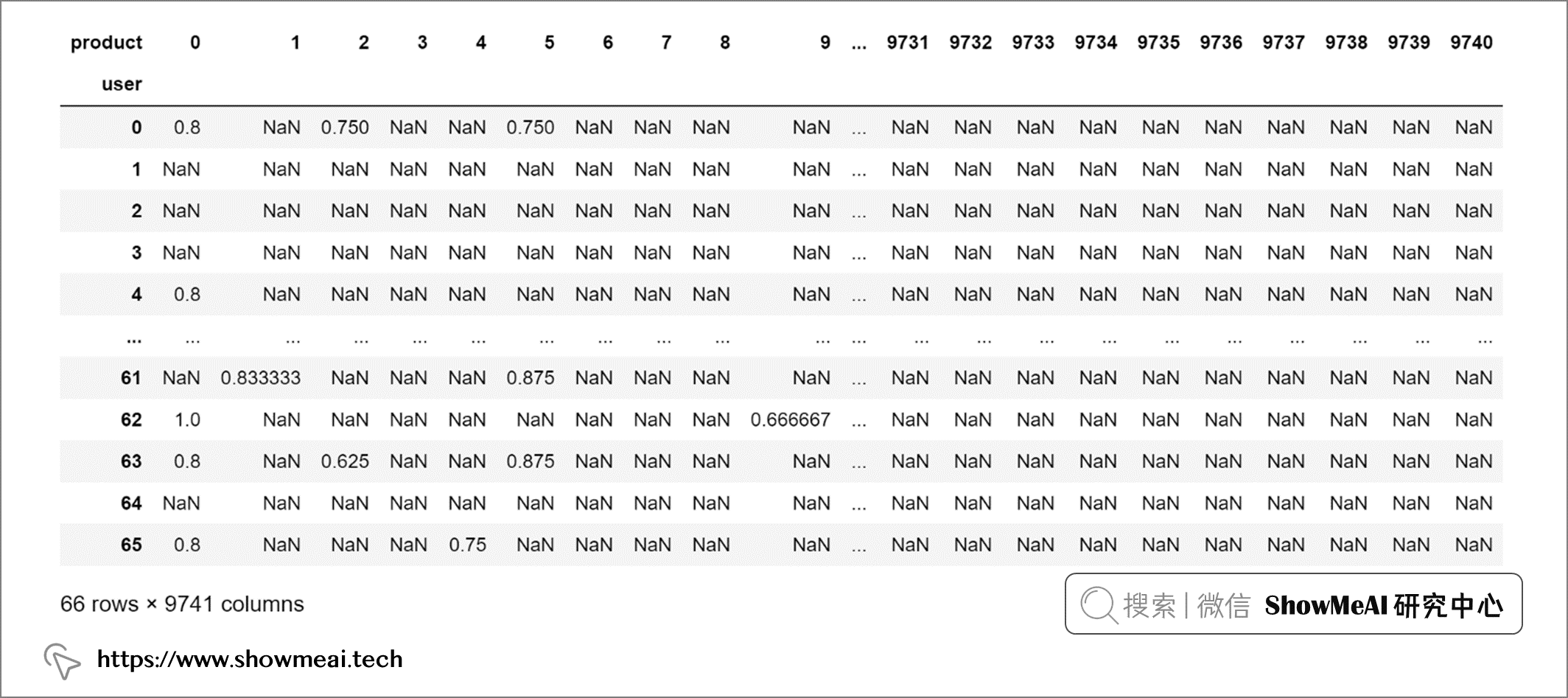## 📌 数据切分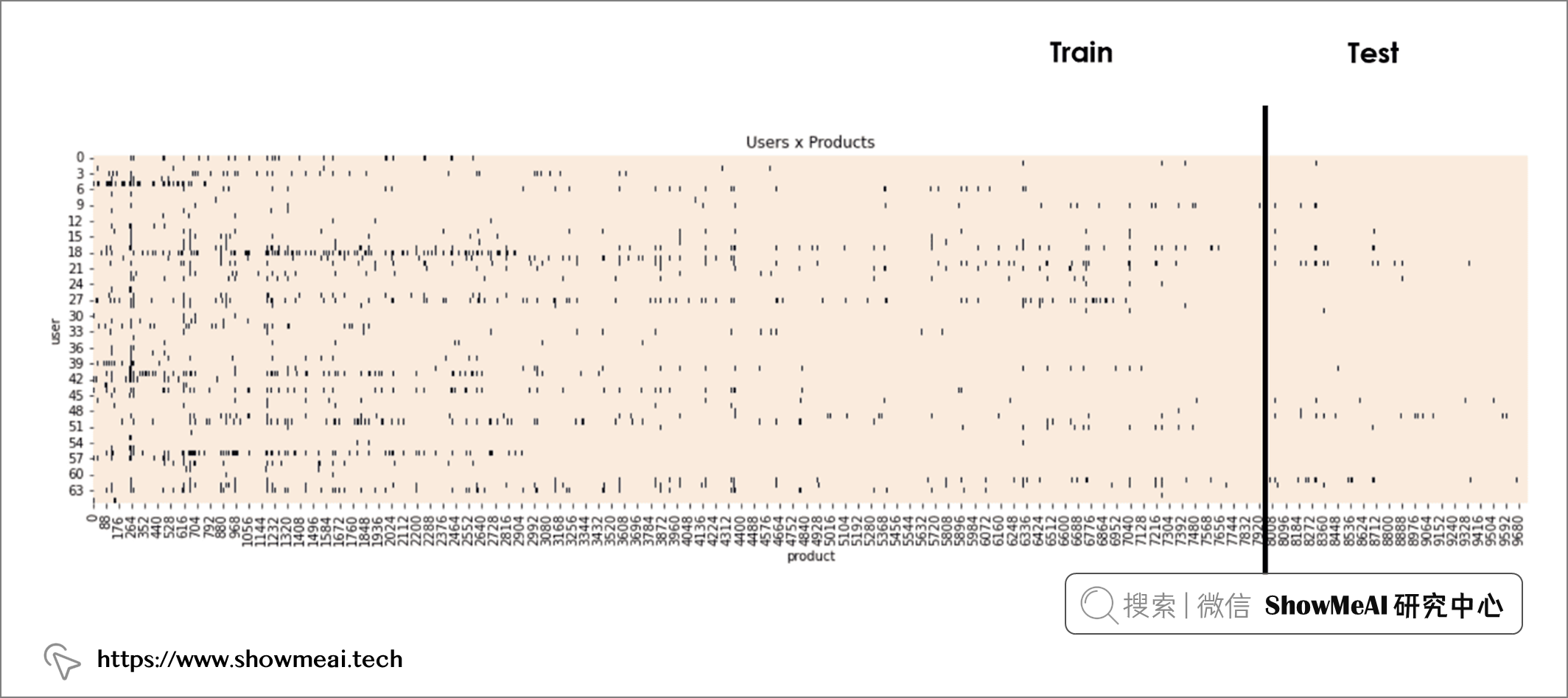# 数据切分
split = int(0.8*dtf_users.shape)
dtf_train = dtf_users.loc[:, :split-1]
dtf_test = dtf_users.loc[:, split:]

# 💡 基于内容的推荐方法

## 📌 核心思想

 ![](https://p3-juejin.byteimg.com/tos-cn-i-k3u1fbpfcp/7f5231b942ca44f5b8c5471a869107cf~tplv-k3u1fbpfcp-zoom-1.image)

## 📌 代码实现

# 选一个user
i = 1
train = dtf_train.iloc[i].to_frame(name="y")
test = dtf_test.iloc[i].to_frame(name="y")

# 把所有测试集的电影评分清空后拼接
tmp = test.copy()
tmp["y"] = np.nan
train = train.append(tmp)

# 数据维度
usr = train[["y"]].fillna(0).values.T
prd = dtf_products.drop(["name","genres"],axis=1).values
print("Users", usr.shape, " x  Products", prd.shape)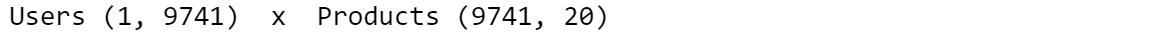# usr_ft(users,fatures) = usr(users,products) x prd(products,features)
usr_ft = np.dot(usr, prd)

# 归一化
weights = usr_ft / usr_ft.sum()

# 预估打分 rating(users,products) = weights(users,fatures) x prd.T(features,products)
pred = np.dot(weights, prd.T)

test = test.merge(pd.DataFrame(pred, columns=["yhat"]), how="left", left_index=True, right_index=True).reset_index()
test = test[~test["y"].isna()]
test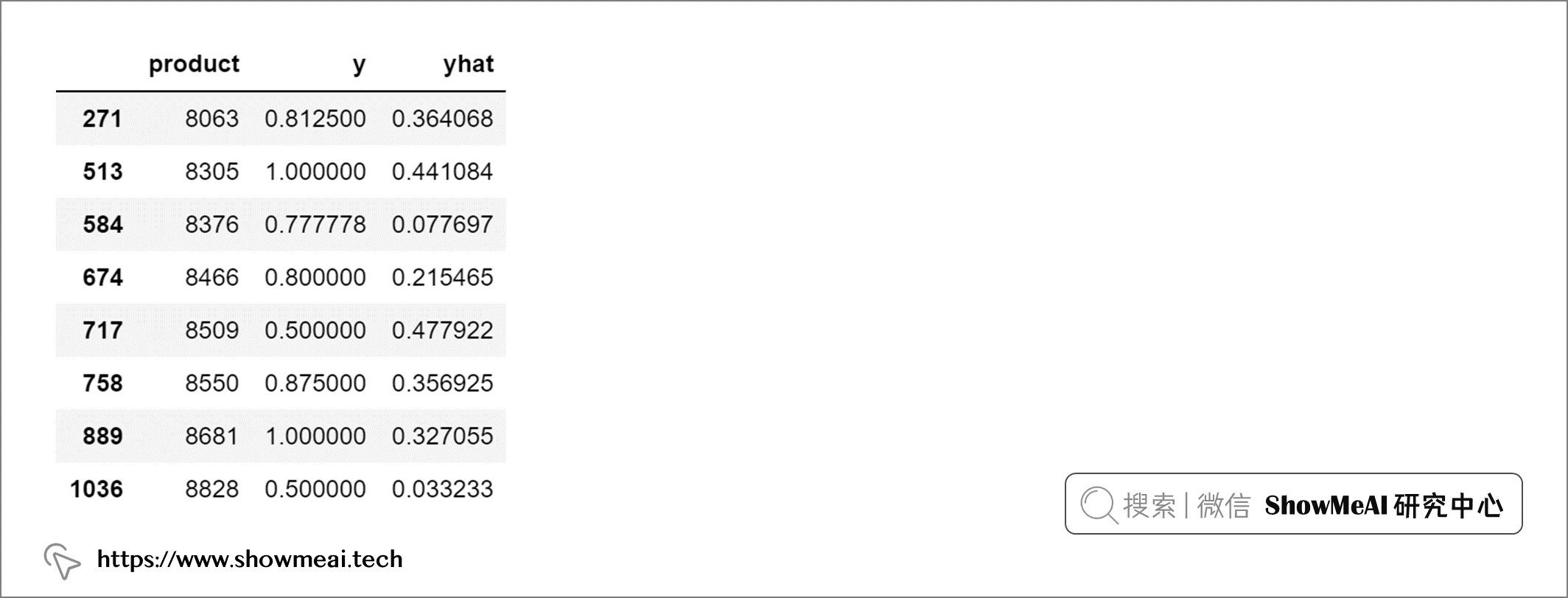# 基于tensorflow更高效的实现
import tensorflow as tf

# usr_ft(users,fatures) = usr(users,products) x prd(products,features)
usr_ft = tf.matmul(usr, prd)

# normalize
weights = usr_ft / tf.reduce_sum(usr_ft, axis=1, keepdims=True)

# rating(users,products) = weights(users,fatures) x prd.T(features,products)
pred = tf.matmul(weights, prd.T)

# 评估指标
def mean_reciprocal_rank(y_test, predicted):
score = []
for product in y_test:
mrr = 1 / (list(predicted).index(product) + 1) if product
in predicted else 0
score.append(mrr)
return np.mean(score)

print("--- user", i, "---")

top = 5
y_test = test.sort_values("y", ascending=False)["product"].values[:top]
print("y_test:", y_test)

predicted = test.sort_values("yhat", ascending=False)["product"].values[:top]
print("predicted:", predicted)

true_positive = len(list(set(y_test) & set(predicted)))
print("true positive:", true_positive, "("+str(round(true_positive/top*100,1))+"%)")
print("accuracy:", str(round(metrics.accuracy_score(y_test,predicted)*100,1))+"%")
print("mrr:", mean_reciprocal_rank(y_test, predicted))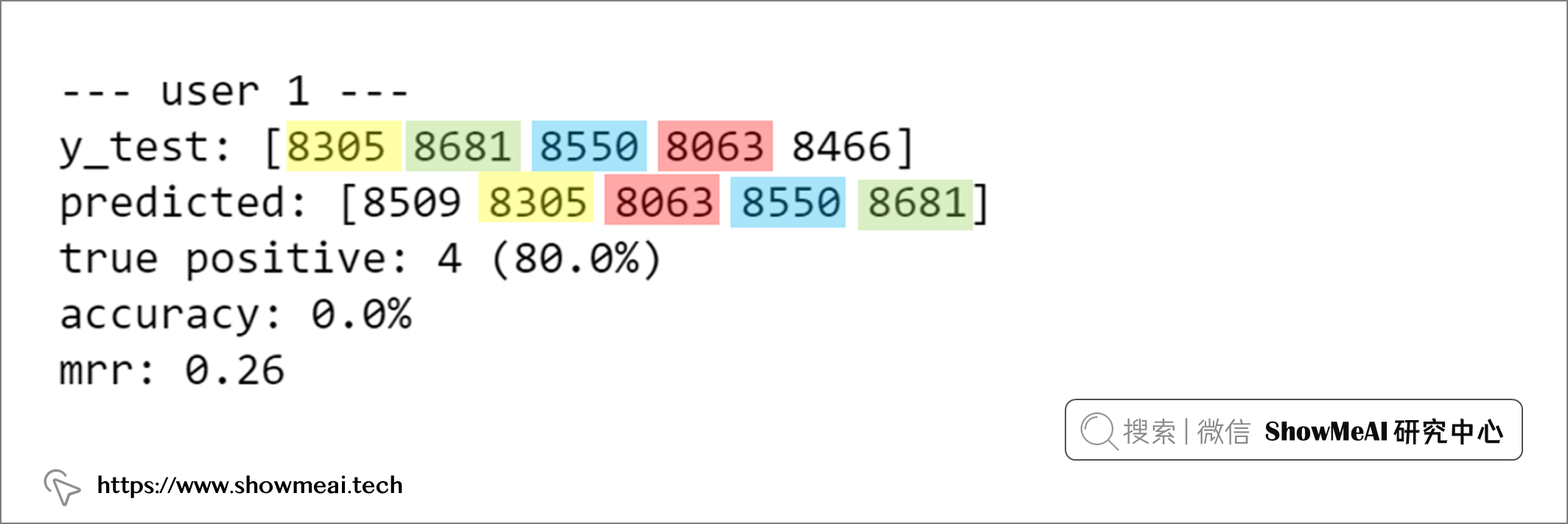# 查看预估结果细节
test.merge(
dtf_products[["name","old","genres"]], left_on="product",
right_index=True
).sort_values("yhat", ascending=False)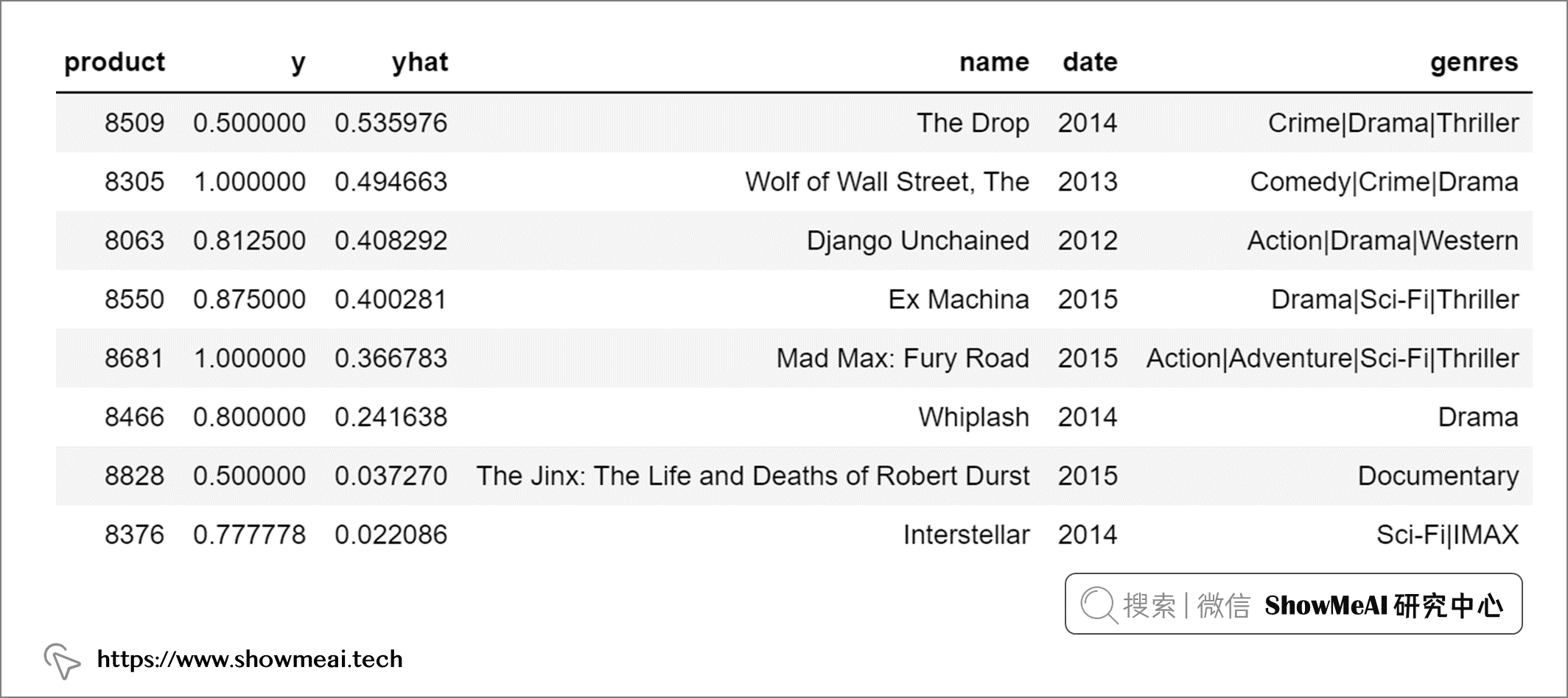# 💡 协同过滤推荐算法

## 📌 核心思想

 ![](https://p3-juejin.byteimg.com/tos-cn-i-k3u1fbpfcp/3be19579b1204da4bc7486f4fac03122~tplv-k3u1fbpfcp-zoom-1.image)

## 📌 基础协同过滤算法

### 基于近邻的协同过滤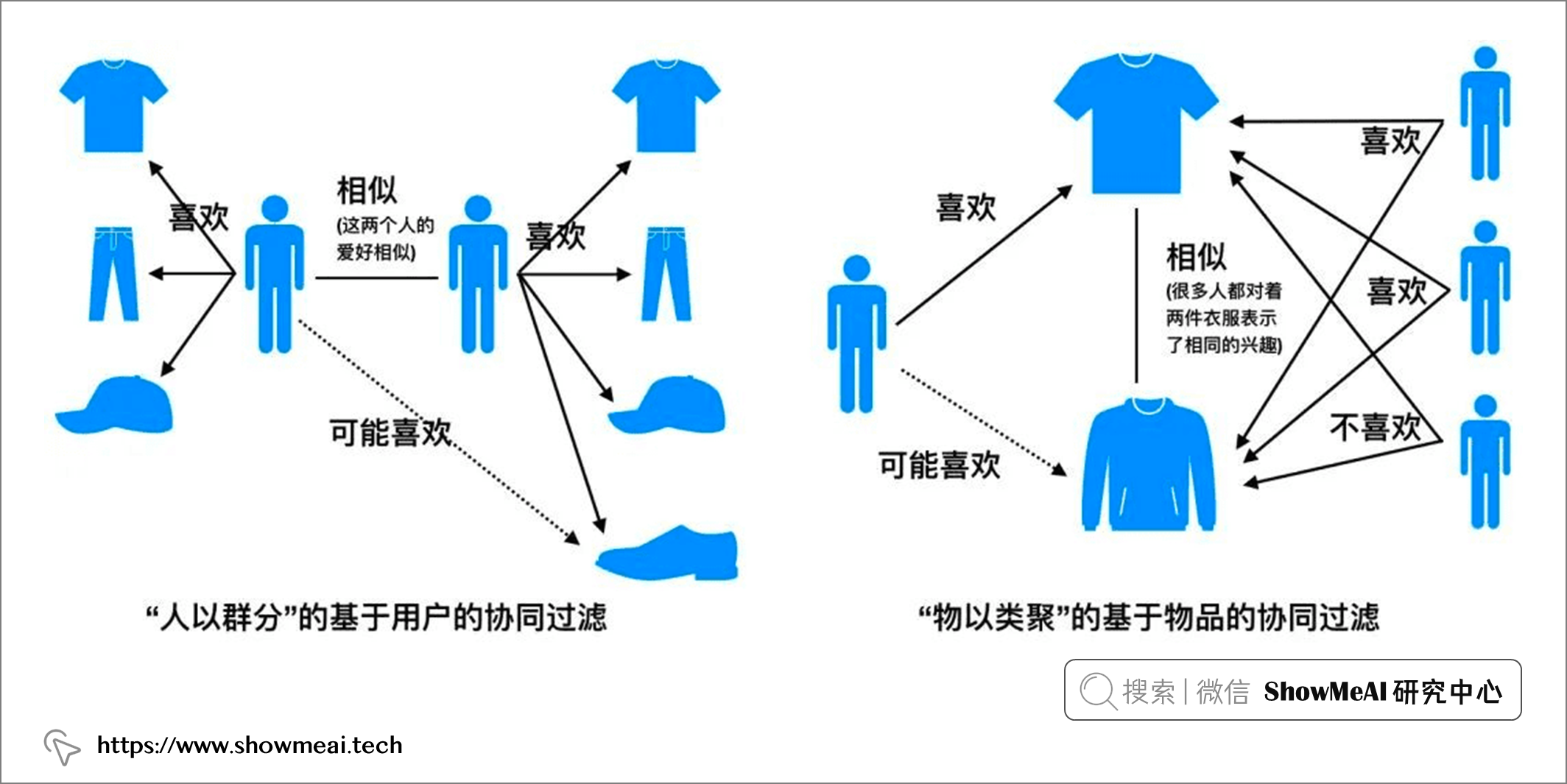① 根据历史数据收集用户偏好（比如本例中的打分，比如）。

② 找到相似的用户（基于用户）或物品（基于物品）。

③ 基于相似性计算和推荐。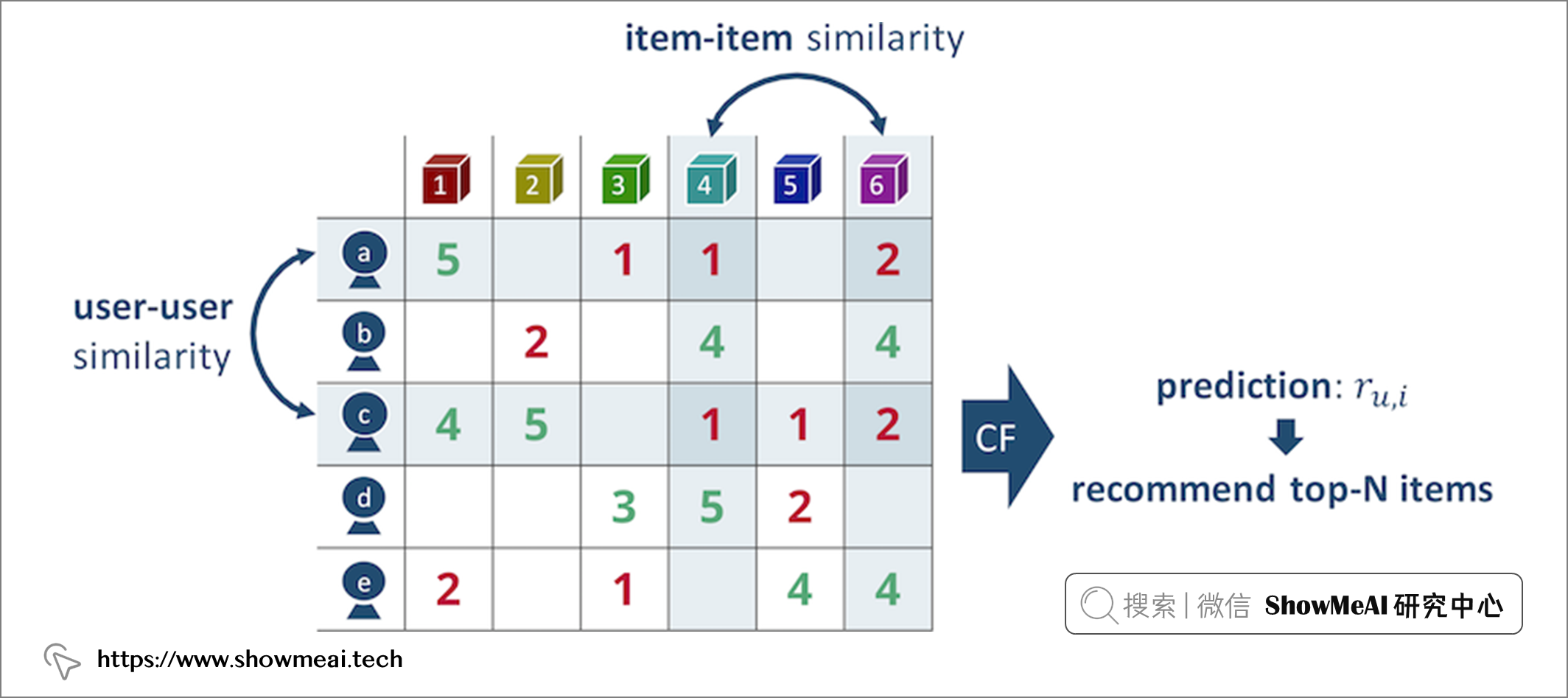$$\text{cosine similarity}=S_{C}(A, B):=\cos (\theta)=\frac{A \cdot \mathbf{B}}{\|\mathbf{A}\|\|\mathbf{B}\|}=\frac{\sum_{i=1}^{n} A_{i} B_{i}}{\sqrt{\sum_{i=1}^{n} A_{i}^{2} \sqrt{\sum_{i=1}^{n} B_{i}^{2}}}}$$

### 基于隐语义模型的协同过滤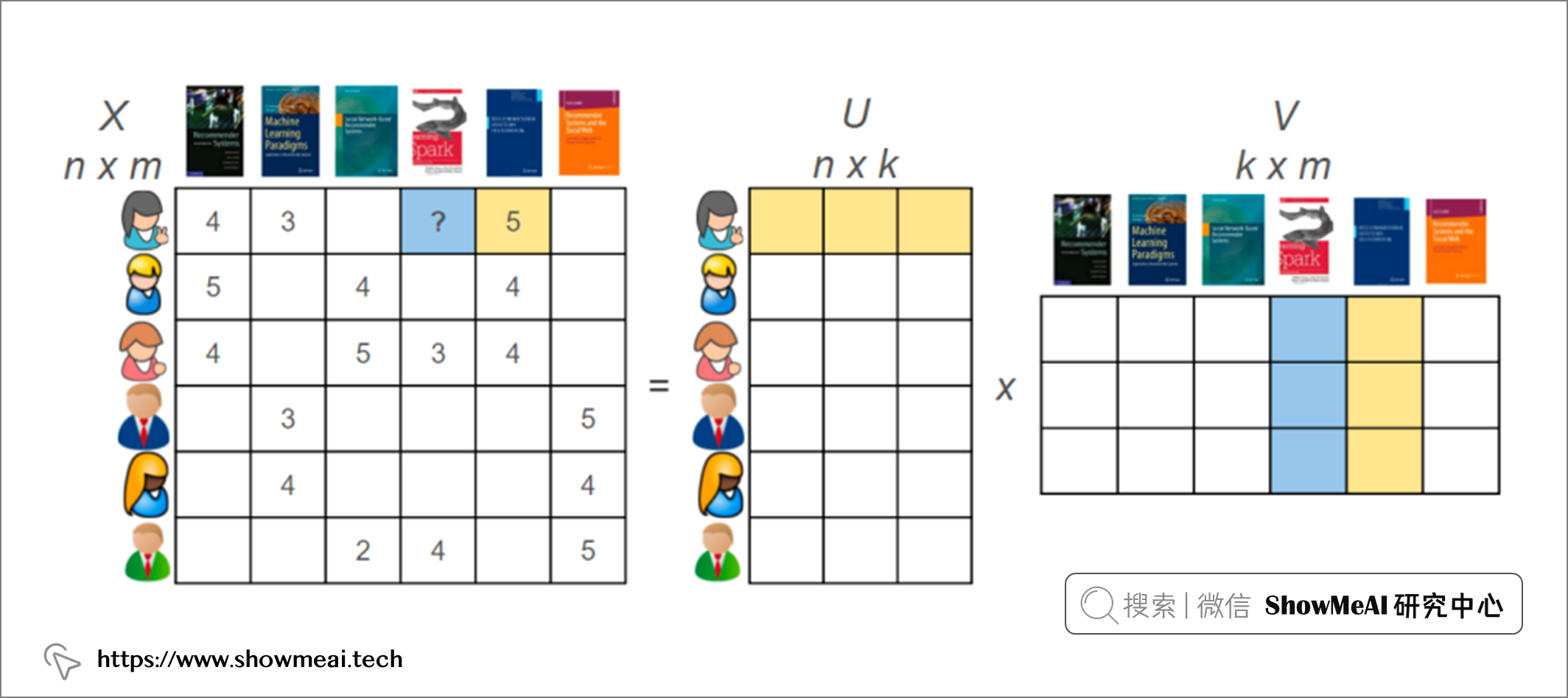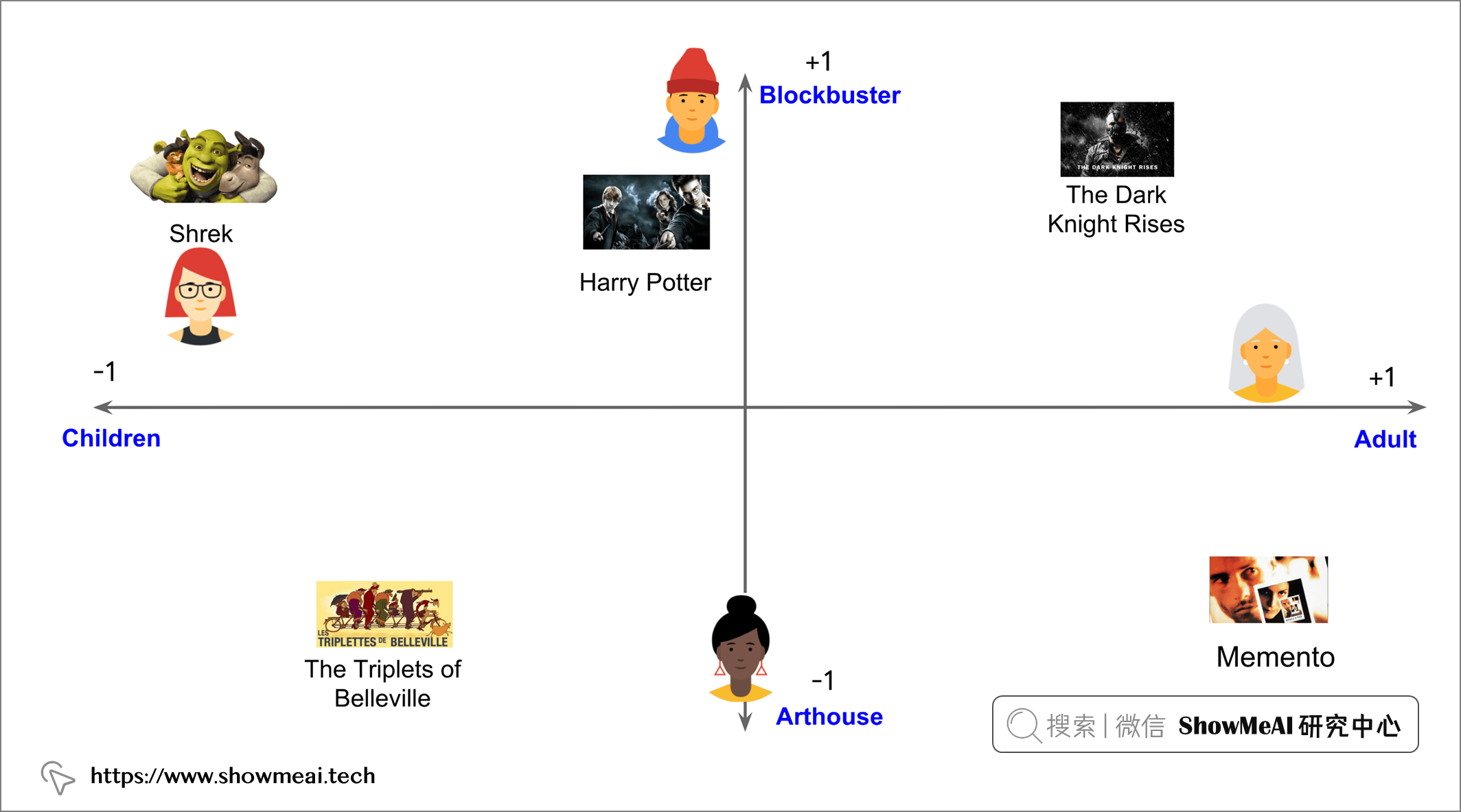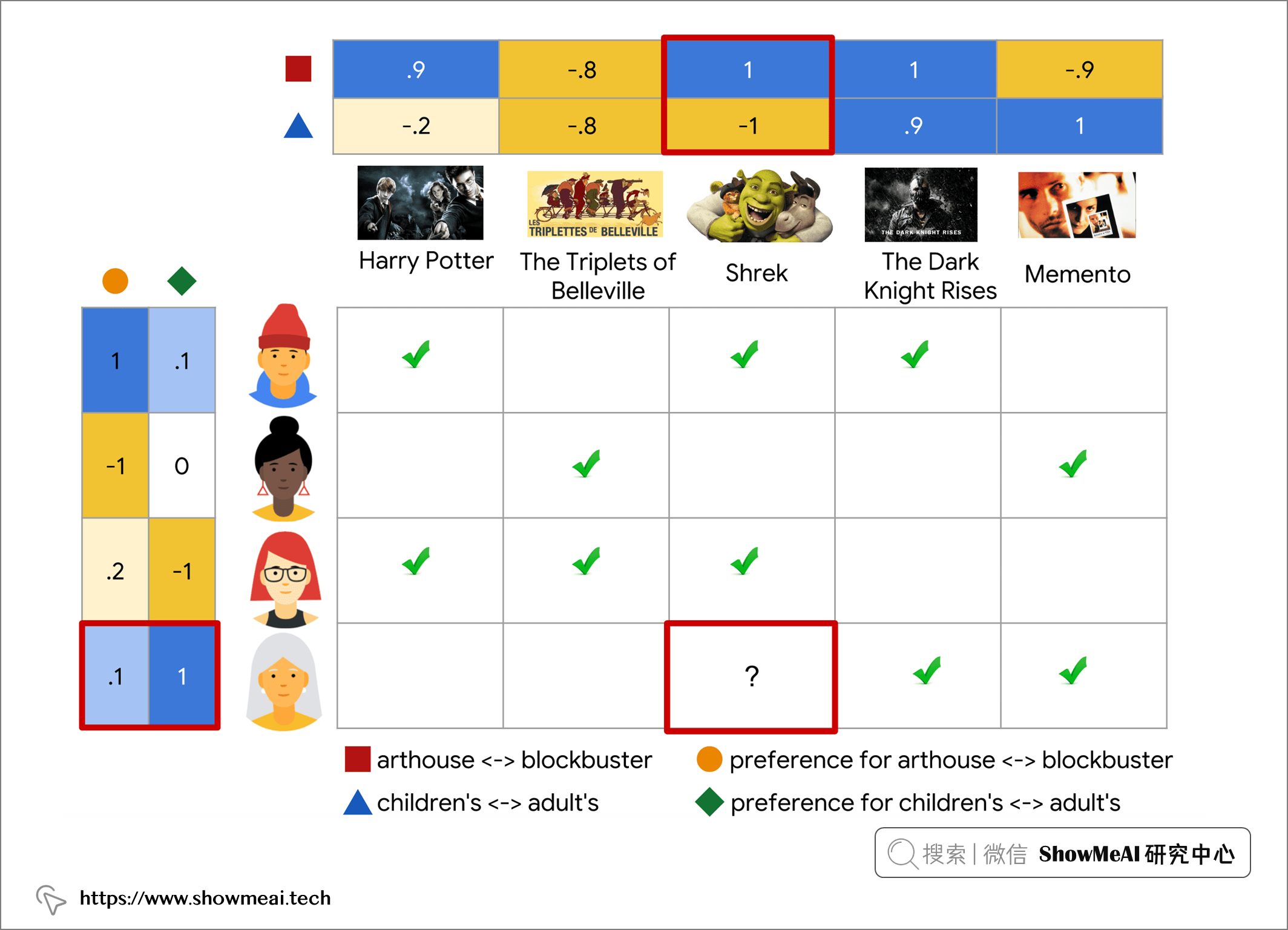### 代码实现

train = dtf_train.stack(dropna=True).reset_index().rename(columns={0:"y"})
train.head()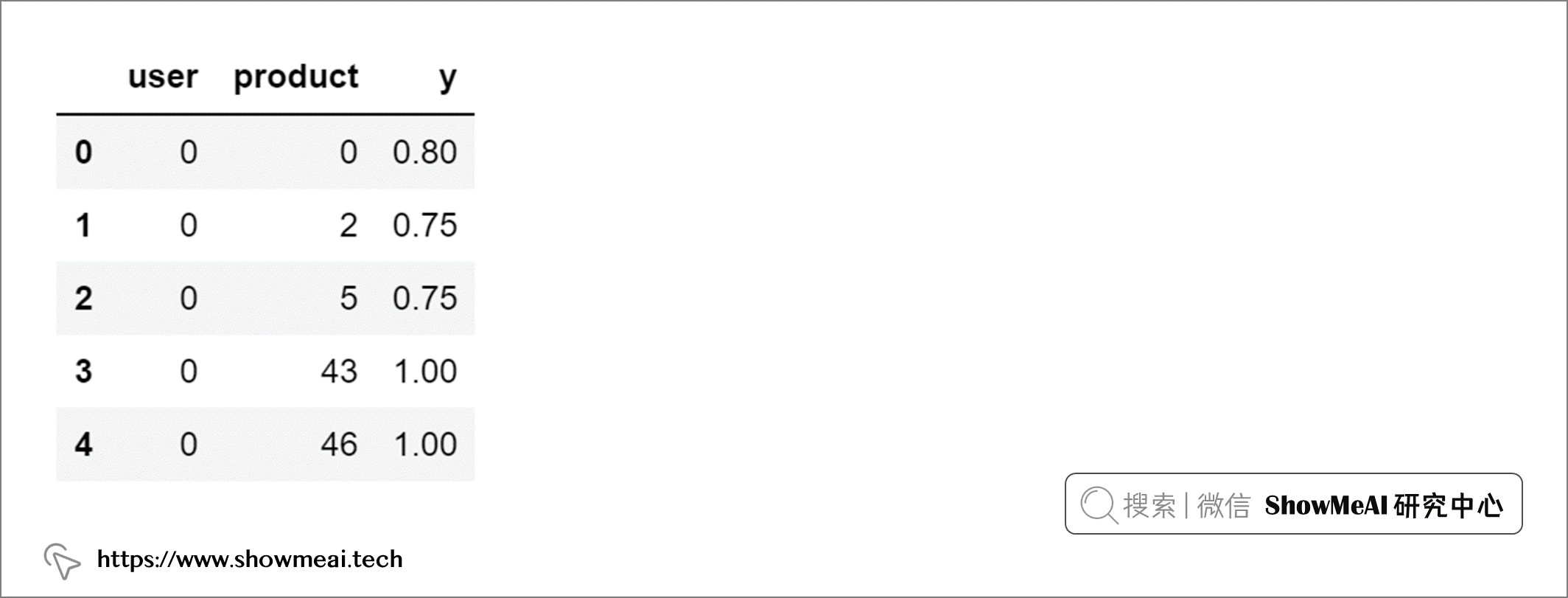embeddings_size = 50
usr, prd = dtf_users.shape, dtf_users.shape

# 用户 Users 维度(1,embedding_size)
xusers_in = layers.Input(name="xusers_in", shape=(1,))
xusers_emb = layers.Embedding(name="xusers_emb", input_dim=usr, output_dim=embeddings_size)(xusers_in)
xusers = layers.Reshape(name='xusers', target_shape=(embeddings_size,))(xusers_emb)

# 产品 Products 维度(1,embedding_size)
xproducts_in = layers.Input(name="xproducts_in", shape=(1,))
xproducts_emb = layers.Embedding(name="xproducts_emb", input_dim=prd, output_dim=embeddings_size)(xproducts_in)
xproducts = layers.Reshape(name='xproducts', target_shape=(embeddings_size,))(xproducts_emb)

# 矩阵乘法，即我们我们上面提到的因子矩阵相乘 维度(1)
xx = layers.Dot(name='xx', normalize=True, axes=1)([xusers, xproducts])

# 预测得分 维度(1)
y_out = layers.Dense(name="y_out", units=1, activation='linear')(xx)

# 编译
model = models.Model(inputs=[xusers_in,xproducts_in], outputs=y_out, name="CollaborativeFiltering")
model.compile(optimizer='adam', loss='mean_absolute_error', metrics=['mean_absolute_percentage_error'])

utils.plot_model(model, to_file='model.png', show_shapes=True, show_layer_names=True)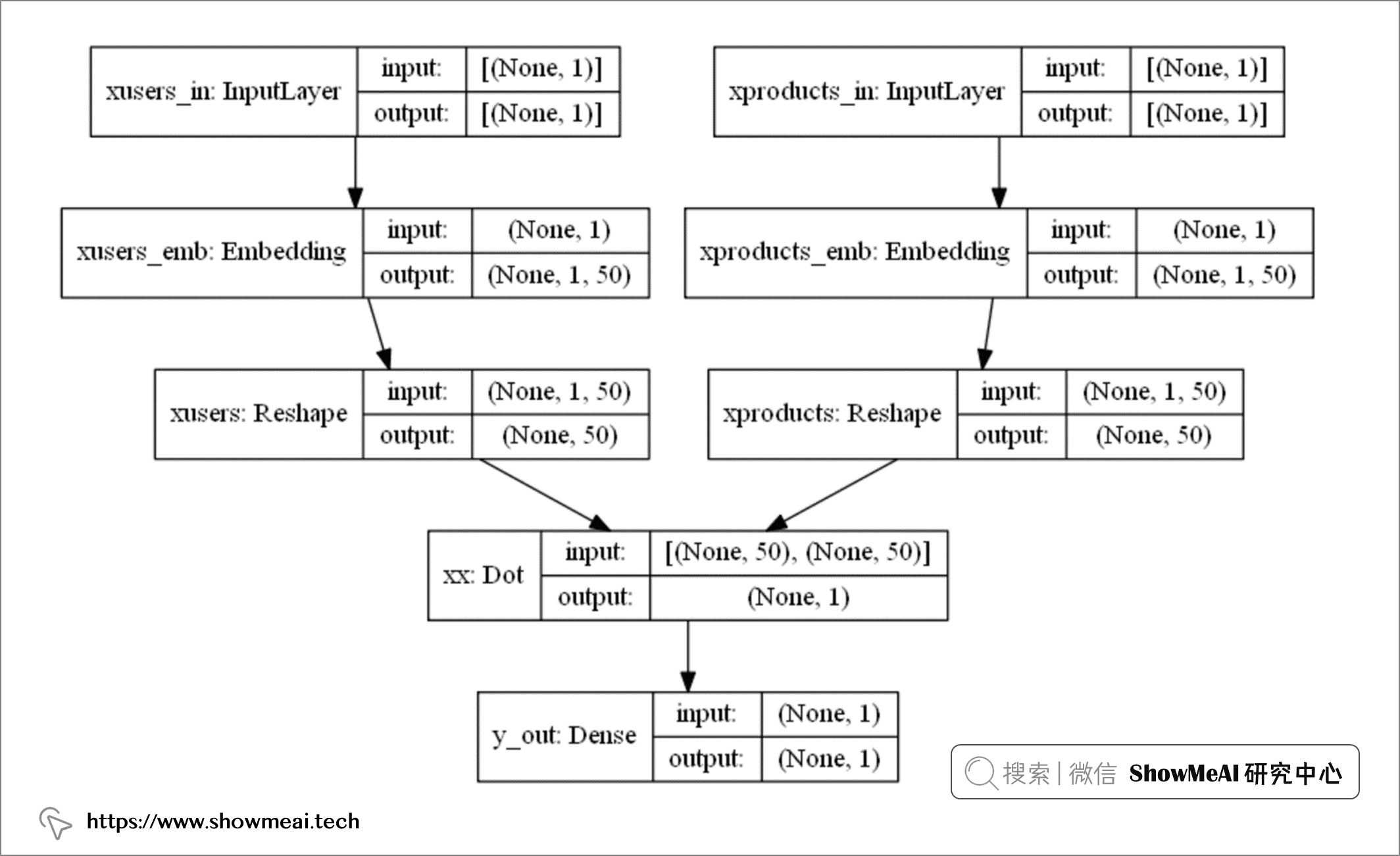# 训练
training = model.fit(x=[train["user"], train["product"]], y=train["y"], epochs=100, batch_size=128, shuffle=True, verbose=0, validation_split=0.3)

model = training.model

# 测试
test["yhat"] = model.predict([test["user"], test["product"]])
test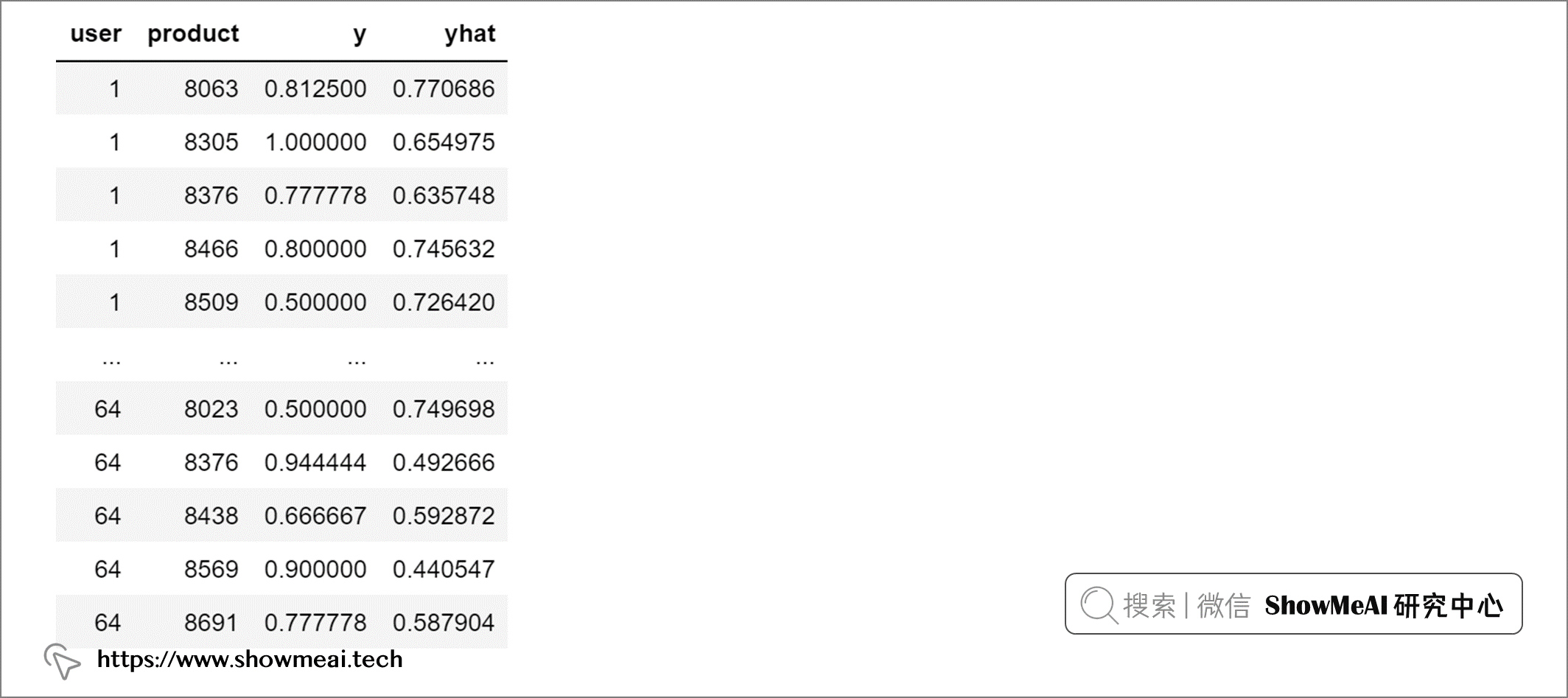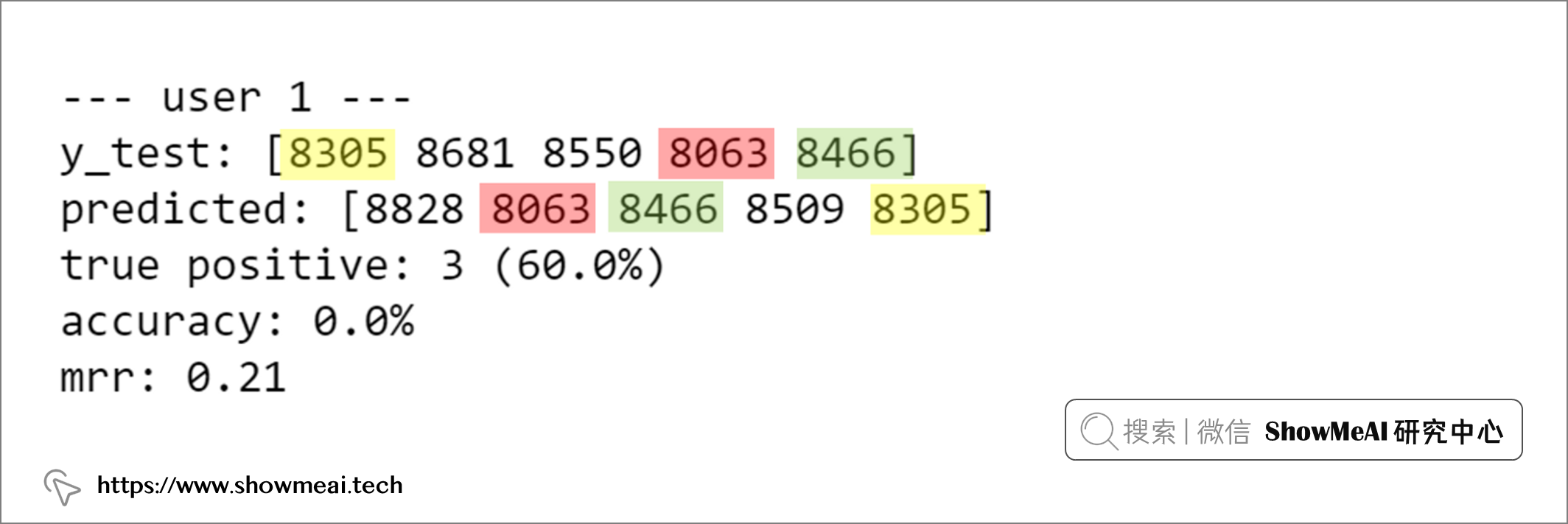## 📌 神经协同过滤算法

### 模型介绍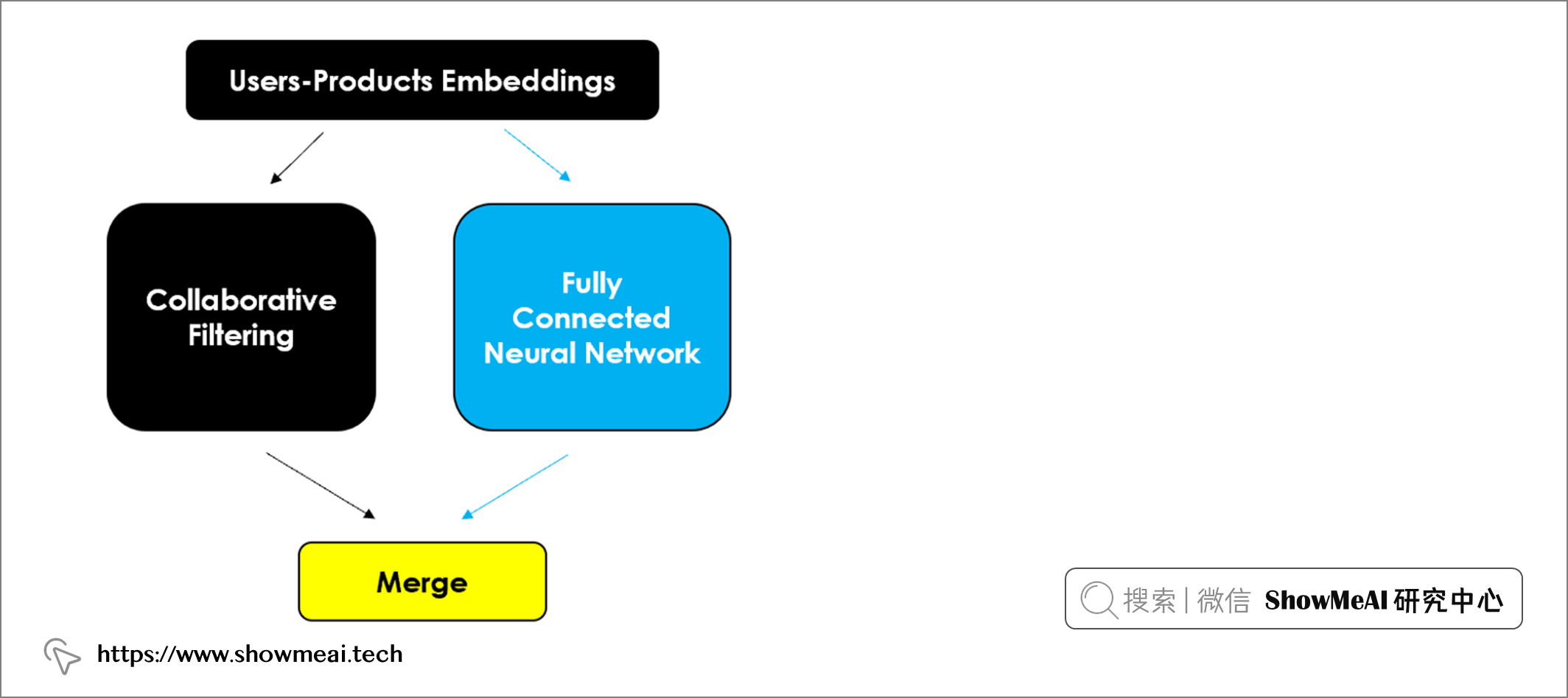### 代码实现

# 用户与产品的embedding维度，相当于协同过滤中的因子数
embeddings_size = 50
usr, prd = dtf_users.shape, dtf_users.shape

# 输入层
xusers_in = layers.Input(name="xusers_in", shape=(1,))
xproducts_in = layers.Input(name="xproducts_in", shape=(1,))

# A) 模型左侧：Matrix Factorization 矩阵分解
# embeddings 与 reshape
cf_xusers_emb = layers.Embedding(name="cf_xusers_emb", input_dim=usr, output_dim=embeddings_size)(xusers_in)
cf_xusers = layers.Reshape(name='cf_xusers', target_shape=(embeddings_size,))(cf_xusers_emb)

# embeddings 与 reshape
cf_xproducts_emb = layers.Embedding(name="cf_xproducts_emb", input_dim=prd, output_dim=embeddings_size)(xproducts_in)
cf_xproducts = layers.Reshape(name='cf_xproducts', target_shape=(embeddings_size,))(cf_xproducts_emb)

# 产品 product
cf_xx = layers.Dot(name='cf_xx', normalize=True, axes=1)([cf_xusers, cf_xproducts])

# B) 模型右侧：Neural Network 神经网络
# embeddings 与 reshape
nn_xusers_emb = layers.Embedding(name="nn_xusers_emb", input_dim=usr, output_dim=embeddings_size)(xusers_in)
nn_xusers = layers.Reshape(name='nn_xusers', target_shape=(embeddings_size,))(nn_xusers_emb)

# embeddings 与 reshape
nn_xproducts_emb = layers.Embedding(name="nn_xproducts_emb", input_dim=prd, output_dim=embeddings_size)(xproducts_in)
nn_xproducts = layers.Reshape(name='nn_xproducts', target_shape=(embeddings_size,))(nn_xproducts_emb)

# 拼接与全连接处理
nn_xx = layers.Concatenate()([nn_xusers, nn_xproducts])
nn_xx = layers.Dense(name="nn_xx", units=int(embeddings_size/2), activation='relu')(nn_xx)

# 合并A和B
y_out = layers.Concatenate()([cf_xx, nn_xx])
y_out = layers.Dense(name="y_out", units=1, activation='linear')(y_out)

# 编译
model = models.Model(inputs=[xusers_in,xproducts_in], outputs=y_out, name="Neural_CollaborativeFiltering")
model.compile(optimizer='adam', loss='mean_absolute_error', metrics=['mean_absolute_percentage_error']) 

utils.plot_model(model, to_file=’model.png’, show_shapes=True, show_layer_names=True)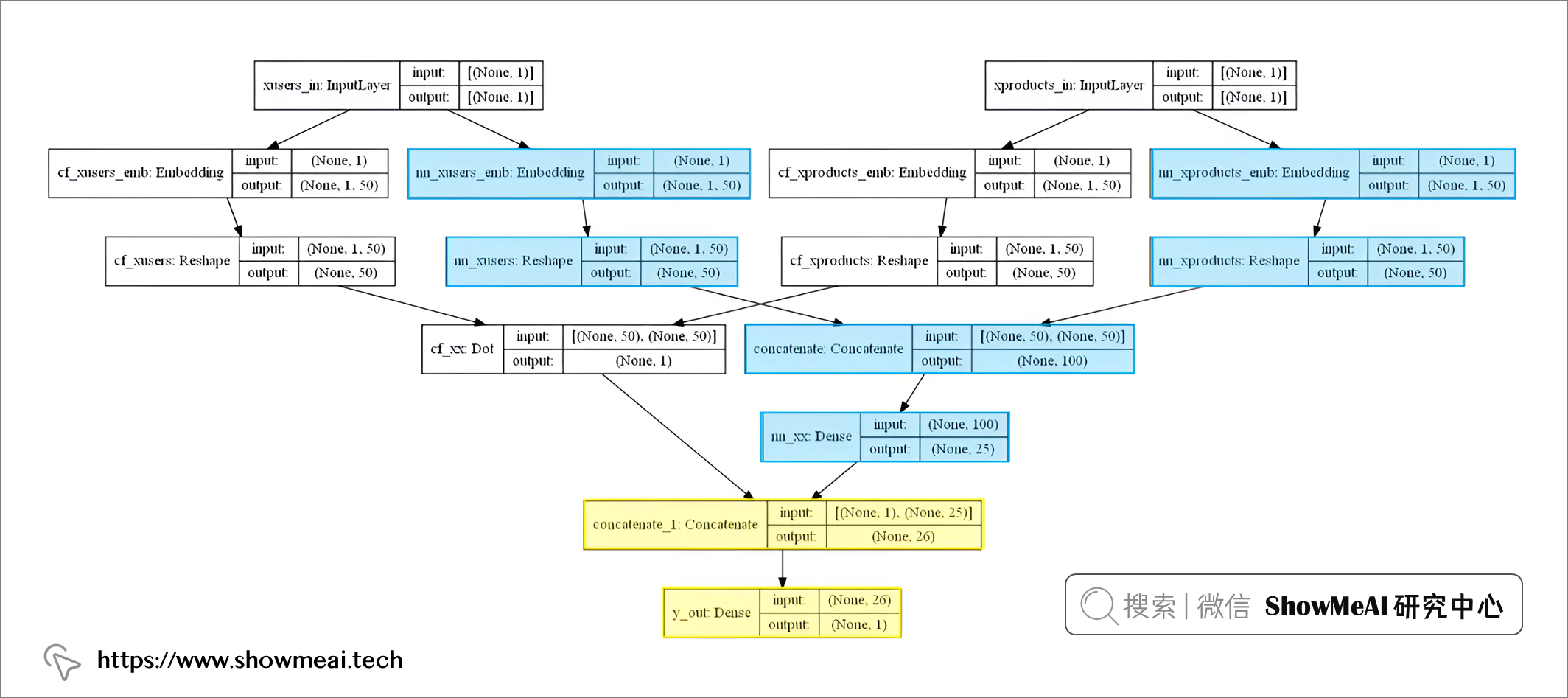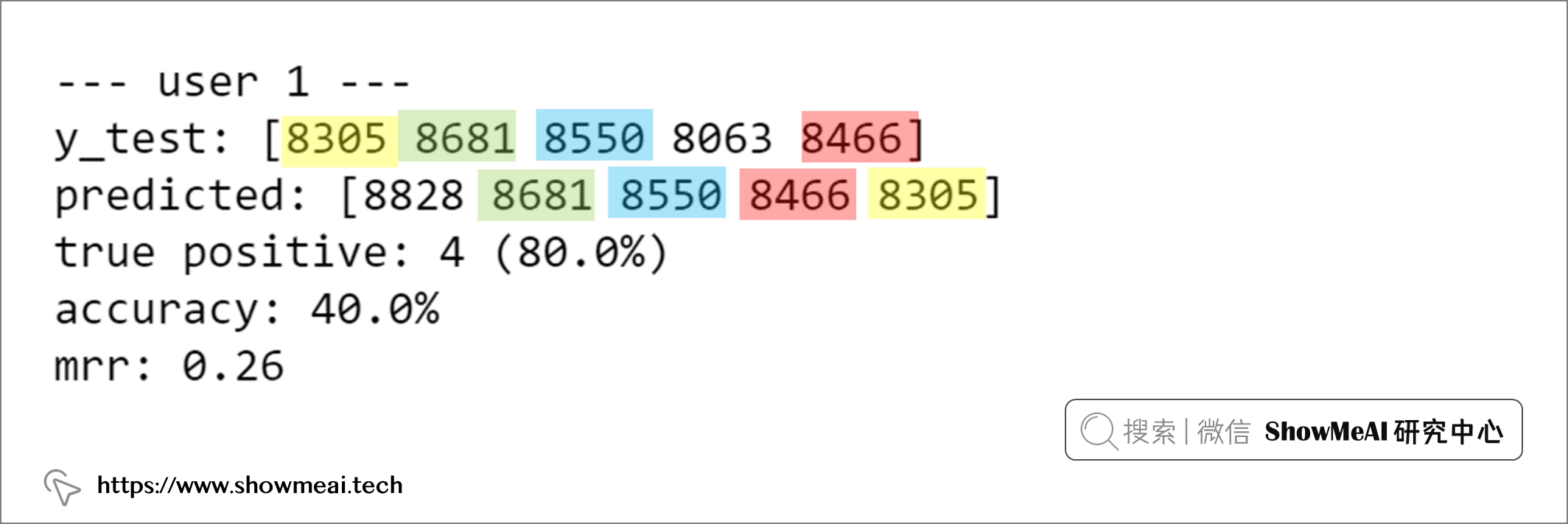# 💡 混合网络模型

## 📌 模型介绍

• 用户行为 当前场景下是电影的打分，它是一种显式用户反馈；有些场景下我们会使用隐式的用户反馈，比如说用户的点击或者深度浏览和完播等行为。
• 产品信息 产品的标签和描述（这里的电影题材、标题等），主要用于基于内容的方法。
• 用户信息 人口统计学信息（即性别和年龄）或行为（即偏好、屏幕上的平均时间、最频繁的使用时间），主要用于基于知识的推荐。
• 上下文 关于评分情况的附加信息（如何时、何地、搜索历史），通常也包含在基于知识的推荐中。

## 📌 代码实现

# 基础特征
features = dtf_products.drop(["genres","name"], axis=1).columns
print(features)

# 上下文特征（时间段、工作日、周末等）
context = dtf_context.drop(["user","product"], axis=1).columns
print(context)train = dtf_train.stack(dropna=True).reset_index().rename(columns={0:"y"})

# 添加特征
train = train.merge(dtf_products[features], how="left", left_on="product", right_index=True)

# 添加上下文信息
train = train.merge(dtf_context, how="left")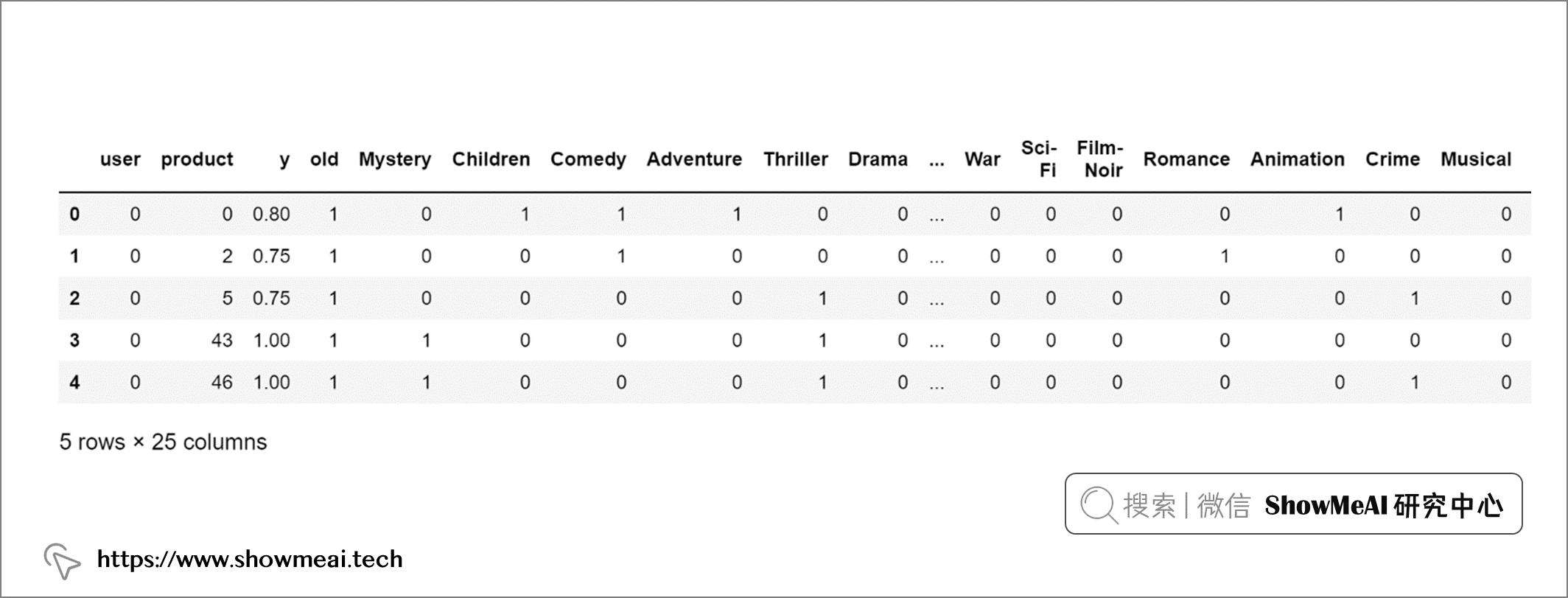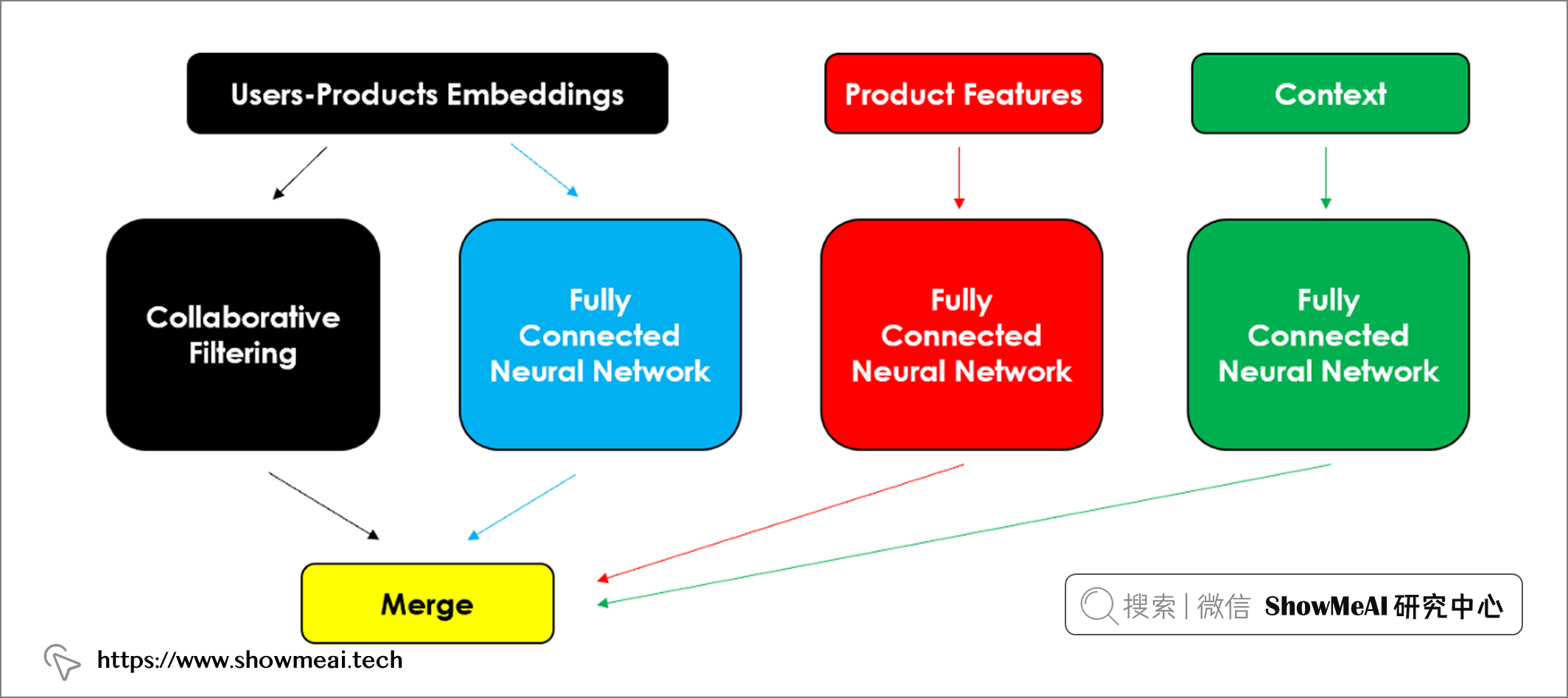embeddings_size = 50
usr, prd = dtf_users.shape, dtf_users.shape
feat = len(features)
ctx = len(context)

################## 神经协同过滤 ########################
# 输入层
xusers_in = layers.Input(name="xusers_in", shape=(1,))
xproducts_in = layers.Input(name="xproducts_in", shape=(1,))

# A) 模型左侧：Matrix Factorization 矩阵分解
# embeddings 与 reshape
cf_xusers_emb = layers.Embedding(name="cf_xusers_emb", input_dim=usr, output_dim=embeddings_size)(xusers_in)
cf_xusers = layers.Reshape(name='cf_xusers', target_shape=(embeddings_size,))(cf_xusers_emb)

# embeddings 与 reshape
cf_xproducts_emb = layers.Embedding(name="cf_xproducts_emb", input_dim=prd, output_dim=embeddings_size)(xproducts_in)
cf_xproducts = layers.Reshape(name='cf_xproducts', target_shape=(embeddings_size,))(cf_xproducts_emb)

# 产品 product
cf_xx = layers.Dot(name='cf_xx', normalize=True, axes=1)([cf_xusers, cf_xproducts])

# B) 模型右侧：Neural Network 神经网络
# embeddings 与 reshape
nn_xusers_emb = layers.Embedding(name="nn_xusers_emb", input_dim=usr, output_dim=embeddings_size)(xusers_in)
nn_xusers = layers.Reshape(name='nn_xusers', target_shape=(embeddings_size,))(nn_xusers_emb)

# embeddings 与 reshape
nn_xproducts_emb = layers.Embedding(name="nn_xproducts_emb", input_dim=prd, output_dim=embeddings_size)(xproducts_in)
nn_xproducts = layers.Reshape(name='nn_xproducts', target_shape=(embeddings_size,))(nn_xproducts_emb)

# 拼接与全连接处理
nn_xx = layers.Concatenate()([nn_xusers, nn_xproducts])
nn_xx = layers.Dense(name="nn_xx", units=int(embeddings_size/2), activation='relu')(nn_xx)

######################## 基础信息 ############################
# 电影特征
features_in = layers.Input(name="features_in", shape=(feat,))
features_x = layers.Dense(name="features_x", units=feat, activation='relu')(features_in)

####################### 上下文特征 ###########################
# 上下文特征
contexts_in = layers.Input(name="contexts_in", shape=(ctx,))
context_x = layers.Dense(name="context_x", units=ctx, activation='relu')(contexts_in)

######################### 输出 ##################################
# 合并所有信息
y_out = layers.Concatenate()([cf_xx, nn_xx, features_x, context_x])
y_out = layers.Dense(name="y_out", units=1, activation='linear')(y_out)

# 编译
model = models.Model(inputs=[xusers_in,xproducts_in, features_in, contexts_in], outputs=y_out, name="Hybrid_Model")
model.compile(optimizer='adam', loss='mean_absolute_error', metrics=['mean_absolute_percentage_error'])

utils.plot_model(model, to_file='model.png', show_shapes=True, show_layer_names=True)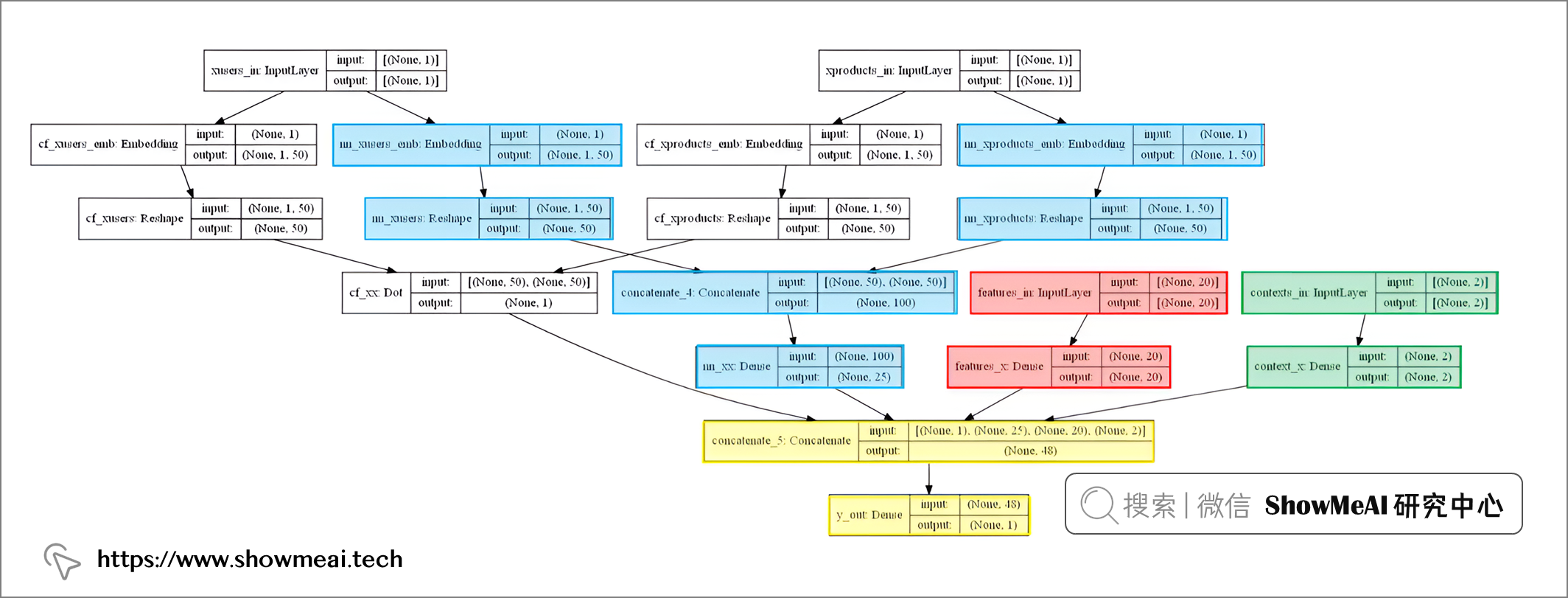# 训练
training = model.fit(x=[train["user"], train["product"], train[features], train[context]], y=train["y"],
epochs=100, batch_size=128, shuffle=True, verbose=0, validation_split=0.3)
model = training.model

# 预测
test["yhat"] = model.predict([test["user"], test["product"], test[features], test[context]])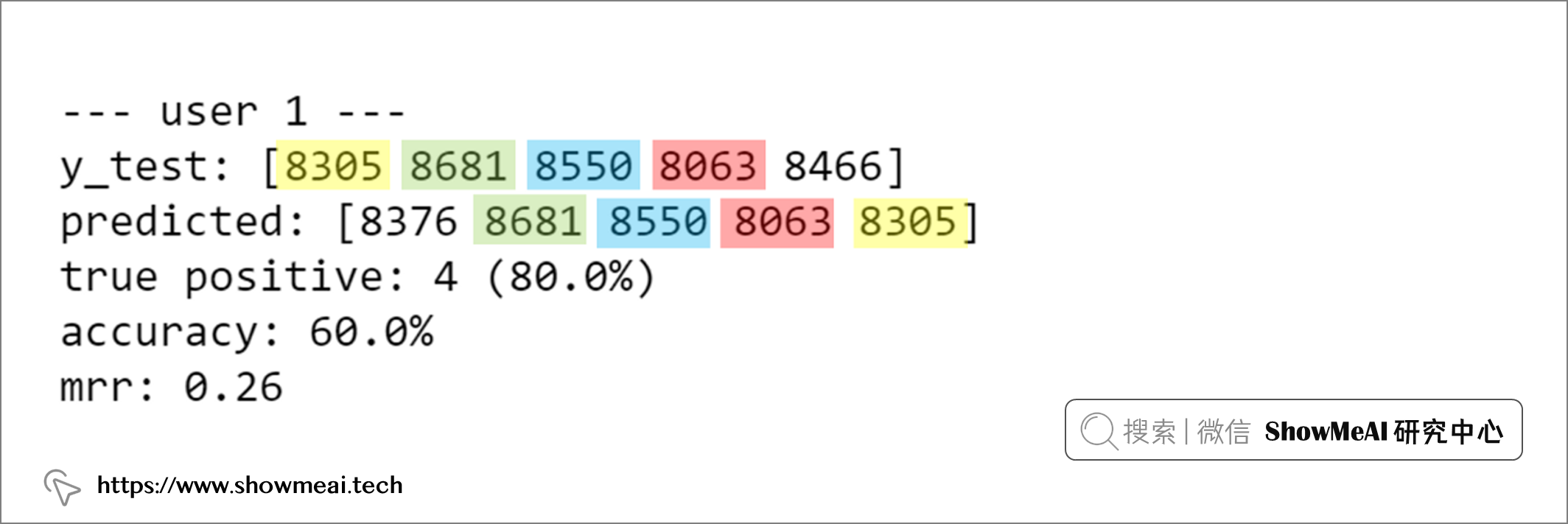# 参考资料|

277 0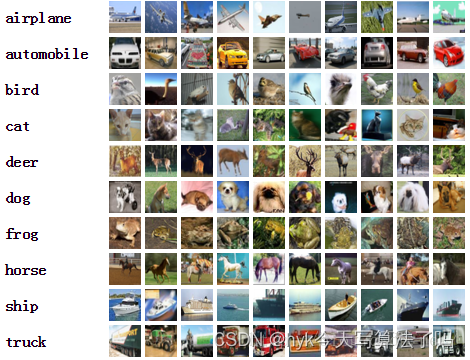|

205 0
|

TensorFlow（1）：使用Docker镜像搭建TensorFlow环境
TensorFlow（1）：使用Docker镜像搭建TensorFlow环境
502 0
|

223 0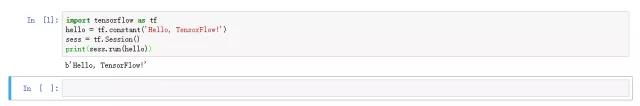|

|

|

1569 0
|
TensorFlow 算法框架/工具 Apache

1375 0
|
TensorFlow 算法框架/工具 机器学习/深度学习

1774 0